Courses
Courses for Kids
Free study material
Offline Centres
More

# NCERT Exemplar for Class 11 Physics Chapter 9 - Mechanical Properties of Solids (Book Solutions)Last updated date: 26th Nov 2023
Total views: 610.5k
Views today: 16.10k

## NCERT Exemplar for Class 11 Physics - Mechanical Properties of Solids - Free PDF Download

Free PDF download of NCERT Exemplar for Class 11 Physics  Chapter 9 - Mechanical Properties of Solids solved by expert Physics teachers on Vedantu.com as per NCERT (CBSE) Book guidelines. All Chapter 9 - Mechanical Properties of Solids Exercise questions with solutions to help you to revise the complete syllabus and score more marks in your Examinations.

The NCERT Exemplar of Physics is suggested by CBSE. The books include all the important topics and concepts that the students of Class 11 need to understand. It will help them to pursue their future academic career. The talented students who aspire to qualify for JEE Main or Advanced and other engineering Examinations can consult these books for better understanding. All the NCERT Exemplars are available on the website of Vedantu. The course content along with all the important topics have been assembled in one place so that the students do not need to undergo the hassle of collecting information from various sources.

## Access NCERT Exemplar Solutions for Class 11 Science (Physics) Chapter 9 – Mechanical Properties Of Solids

### Multiple Choice Questions – I

1. Modulus of rigidity of ideal liquids is

(a) infinity.

(b) zero.

(c) unity.

(d) some finite small non-zero constant value.

Ans: Correct option (b) because since we know from a given conditions that liquid is ideal, thus it does not have frictional force among its layers. Hence, tangential forces are zero since no stress is developed there.

1. The maximum load a wire can withstand without breaking when its length is reduced to half of its original length, will

(a) be double.

(b) be half.

(c) be four times.

(d) remain same.

Ans: Correct option (d) because Breaking stress ${\text{ = }}\dfrac{{{\text{Breaking force}}}}{{{\text{Area of crosss ection}}}}$

As breaking force does not depend on length. Thus, changing the cross section has no effect which implies breaking force remain same.

1. The temperature of a wire is doubled. The Young's modulus of elasticity

(a) will also double.

(b) will become four times.

(c ) will remain same.

(d) will decrease.

Ans: Correct option (d) because as we know that ${{\text{L}}_{\text{t}}}{\text{ = }}{{\text{L}}_{\text{0}}}{\text{(1 + α }\Delta{ t)}}$

${\text{}\Delta{ L = }}{{\text{L}}_{\text{t}}}{\text{ - }}{{\text{L}}_{\text{0}}}{\text{ = α }}{{\text{L}}_{\text{0}}}{\text{}\Delta{ t}}$

${\text{Y = }}\dfrac{{{\text{stress}}}}{{{\text{ strain }}}}{\text{ = }}\dfrac{{{\text{F}}{{\text{L}}_{\text{0}}}}}{{{\text{A}\Delta{ L}}}}{\text{ = }}\dfrac{{{\text{F}}{{\text{L}}_{\text{0}}}}}{{{\text{A(1 + α }\Delta{ t)}}}}$

${\text{Y}} \propto \dfrac{{\text{1}}}{{{\text{}\Delta{ t}}}}$

Thus, when temperature increases then Young’s modules decreases.

1. A spring is stretched by applying a load to its free end. The strain produced in the spring is

(a) volumetric.

(b) shear.

(c) longitudinal and shear.

(d) longitudinal.

Ans: Correct option is (c) because when spring is stretched by load then its shape and length changes which produces shearing and longitudinal strain.

1. A rigid bar of mass ${\text{M}}$ is supported symmetrically by three wires each of length ${\text{1}}$ . Those at each end are of copper and the middle one is of iron. The ratio of their diameters, if each is to have the same tension, is equal to

(a) $\dfrac{{{{\text{Y}}_{{\text{copper }}}}}}{{{{\text{Y}}_{{\text{iron }}}}}}$

(b) $\sqrt {\dfrac{{{{\text{Y}}_{{\text{iron }}}}}}{{{{\text{Y}}_{{\text{copper }}}}}}}$

(c) $\dfrac{{{{\text{Y}}^2}_{{\text{iron }}}}}{{{{\text{Y}}^2}_{{\text{copper }}}}}$

(d) $\dfrac{{{{\text{Y}}_{{\text{iron }}}}}}{{{{\text{Y}}_{{\text{copper }}}}}}$

Ans: Correct option is (b) because

${\text{Y = }}\dfrac{{{\text{ Stress }}}}{{{\text{ Strain }}}}{\text{ = }}\dfrac{{{\text{FL}}}}{{{\text{A}\Delta{ L}}}}{\text{ = }}\dfrac{{{\text{FL}}}}{{{\text{π }}{{\left( {\dfrac{{\text{D}}}{{\text{2}}}} \right)}^{\text{2}}}{\text{}\Delta{ L}}}}$

${\text{Y = }}\dfrac{{{\text{4FL}}}}{{{\text{π }}{{\text{D}}^{\text{2}}}{\text{}\Delta{ L}}}}$

$\because {\text{ L = l}}$

${\text{}\Delta{ L Copper = }\Delta{ L iron (g)}}$

${\text{F = }}$ weight of rod same in both cases

${\text{Y}} \propto \dfrac{{\text{1}}}{{{{\text{D}}^{\text{2}}}}}$

${{\text{D}}^{\text{2}}} \propto \dfrac{{\text{1}}}{{\text{Y}}}{\text{, D}} \propto \sqrt {\dfrac{{\text{1}}}{{\text{Y}}}}$

$\dfrac{{{{\text{D}}_{{\text{copper }}}}}}{{{{\text{D}}_{{\text{iron }}}}}}{\text{ = }}\sqrt {\dfrac{{{{\text{Y}}_{{\text{iron }}}}}}{{{{\text{Y}}_{{\text{copper }}}}}}}$

1. A mild steel wire of length ${\text{2L}}$ and cross-sectional area A is stretched, well within elastic limit, horizontally between two pillars (Figure). A mass ${\text{m}}$ is suspended from the mid-point of the wire. Strain in the wire is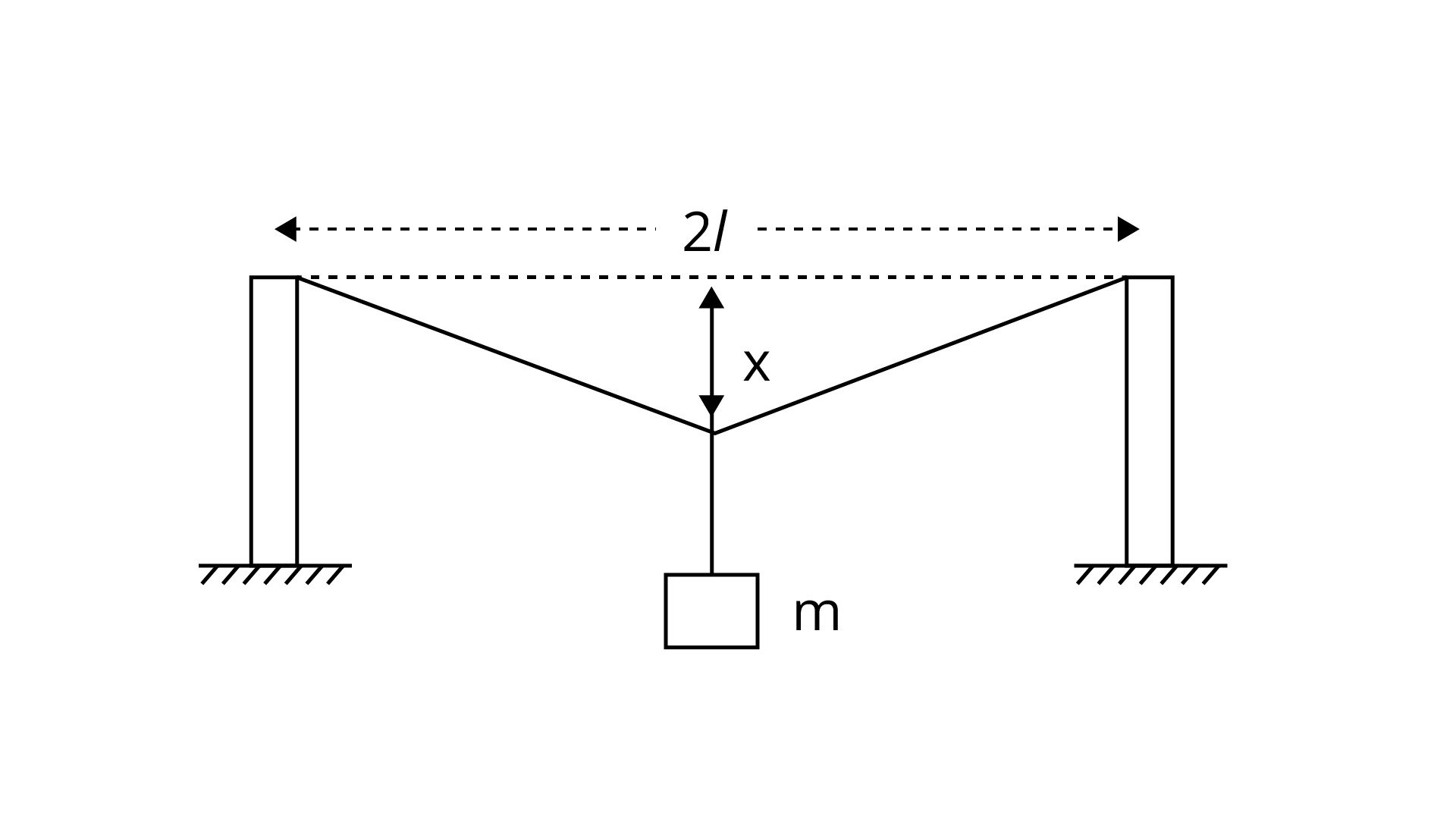(a) $\dfrac{\mathrm{x}^{2}}{2 \mathrm{l}^{2}}$

(b) $\dfrac{x}{1}$

(c) $\dfrac{\mathrm{x}^{2}}{1}$

(d) $\dfrac{\mathrm{x}^{2}}{21}$

Ans: Correct option is (a) because

$\Delta \mathrm{l}=(\mathrm{AO}+\mathrm{BO})-\mathrm{AB}$

$\Delta \mathrm{l}=2 \mathrm{AO}-2 \mathrm{I}=2[\mathrm{AO}-\mathrm{l}]$

$=2\left[\left(\mathrm{l}^{2}+\mathrm{x}^{2}\right)^{\frac{1}{2}}-1\right]$

$=21\left[\left(1+\frac{\mathrm{x}^{2}}{\mathrm{l}^{2}}\right)^{\frac{1}{2}}-1\right]$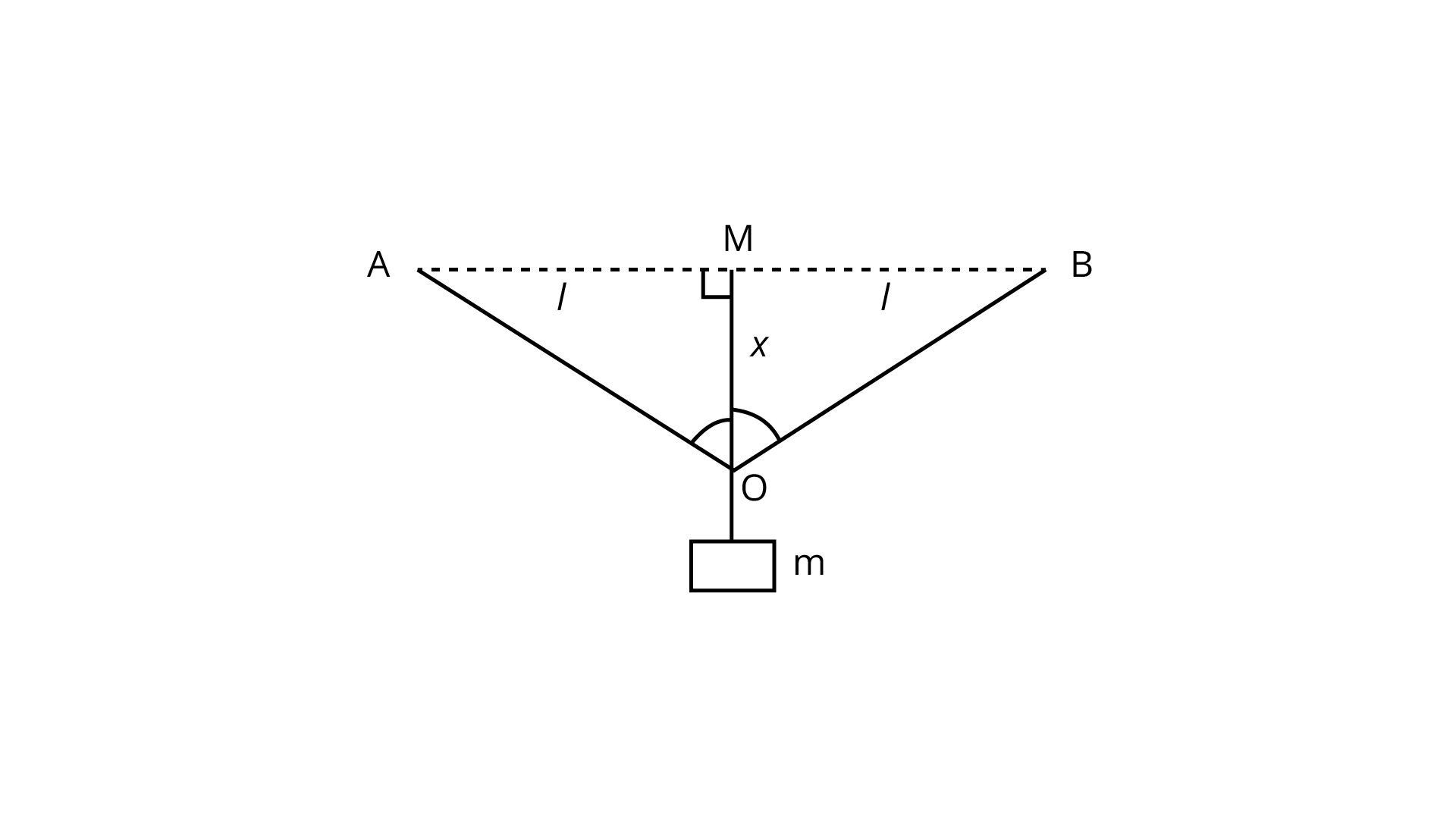$\Delta l=2+\left[1+\frac{x^{2}}{2 l^{2}}-1\right]=2 l \frac{x^{2}}{2 l^{2}} \quad \frac{x^{2}}{l}$

So, strain

$=\frac{\Delta l}{2 l}=\frac{\frac{x^{2}}{1}}{2 l}=\frac{x^{2}}{2 l^{2}}$

1. A rectangular frame is to be suspended symmetrically by two strings of equal length on two supports (Figure). It can be done in one of the following three ways;

(a) The tension in the strings will be

(b) the same in all cases.

(c) least in (a).

(d) least in(c)

Ans: Correct option is (c) because

Let us consider free body diagram since frame is balanced

Therefore, net forces acting on frame is equal to zero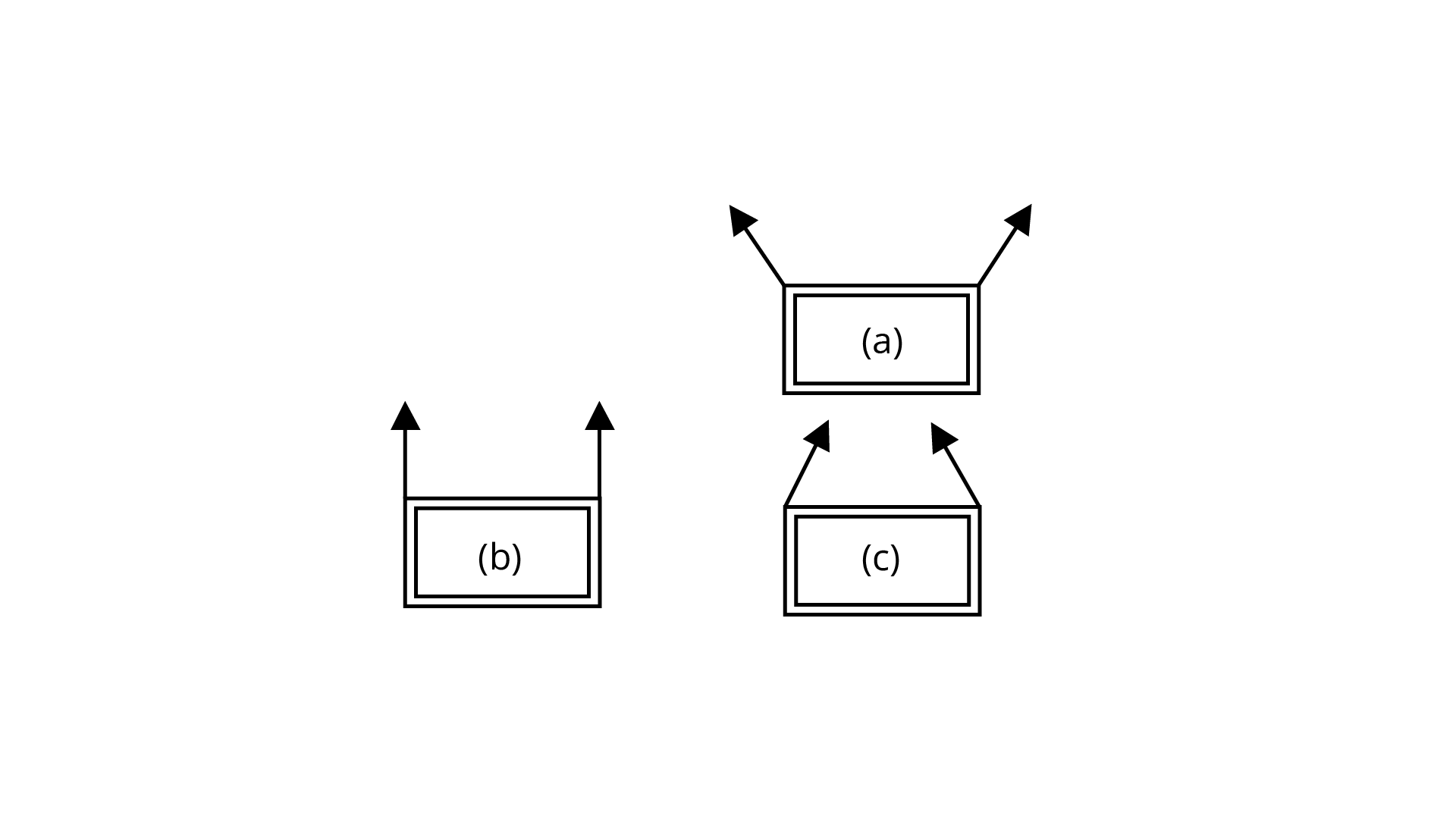Vertical components

${\text{2T sin θ - mg = 0}}$

${\text{2T sin θ = mg}}$

${\text{T = }}\dfrac{{{\text{mg}}}}{{{\text{2 sin θ }}}}$

${\text{T}} \propto \dfrac{{\text{1}}}{{{\text{sin θ }}}}$

So, tensions in all cases are different, for minimum tension ${\text{sinθ }}$ must be ${\text{sinθ = 1 or θ= 9}}{{\text{0}}^\circ}$

1. Consider two cylindrical rods of identical dimensions, one of rubber and the other of steel. Both the rods are fixed rigidly at one end to the roof. A mass ${\text{M}}$ is attached to each of the free ends at the centre of the rods.

(a) Both the rods will elongate but there shall be no perceptible change in shape.

(b) The steel rod will elongate and change shape but the rubber rod will only elongate.

(c) The steel rod will elongate without any perceptible change in shape, but the rubber rod will elongate and the shape of the bottom edge will change to an ellipse.

(d) The steel rod will elongate, without any perceptible change in shape, but the rubber rod will elongate with the shape of the bottom edge tapered to a tip at the centre.

Ans: Correct option is (d) because Mass $\mathrm{M}$ is attached at mid-point of rods of rubber and steel. Since Young's modulus of rigidity for steel is larger than rubber.

Thus, $\frac{\Delta \mathrm{L}}{\mathrm{L}}$ for rubber $\left[\mathrm{Y}=\frac{\frac{\mathrm{F}}{\mathrm{A}}}{\frac{\Delta \mathrm{L}}{\mathrm{L}}}\right]$ is greater than steel.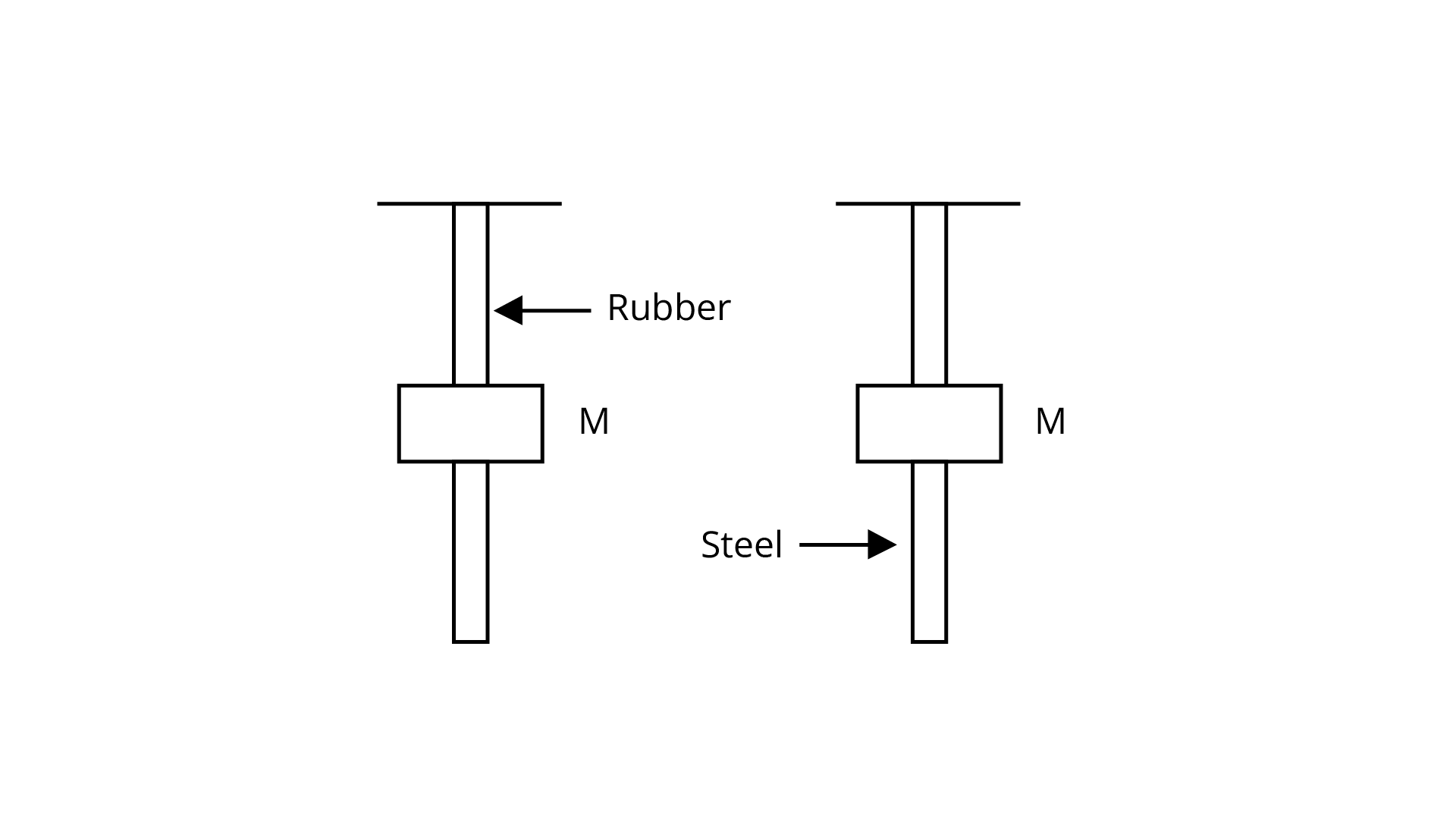Now, in steel rod ${\Delta{ L}}$ is very small and thus it is not visible easily whereas in rubber case, it can be observed early due to changes in its shape.

### Multiple Choice Questions – II

1. The stress-strain graphs for two materials are shown in figure (assume same scale)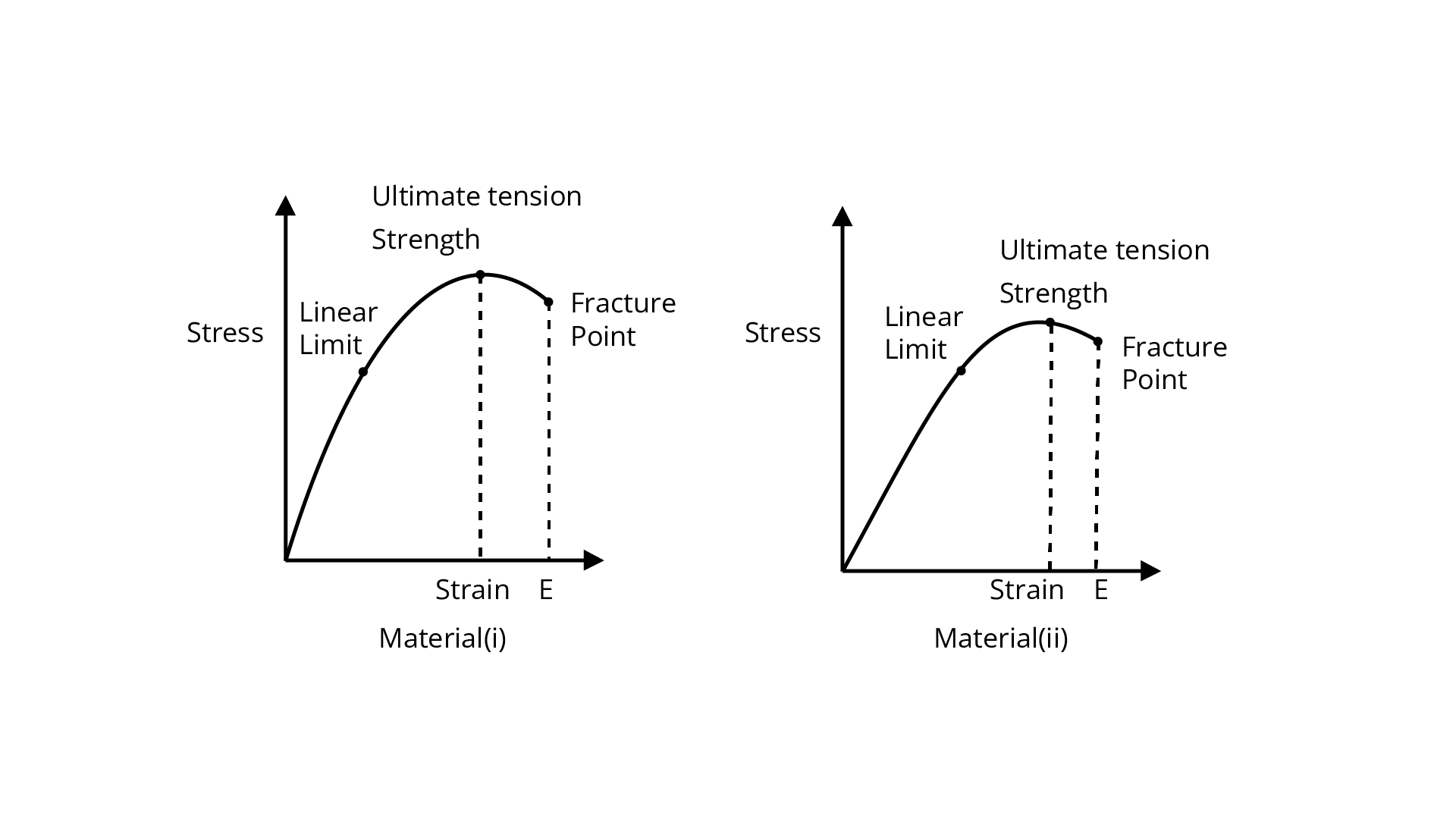(a) Material (ii) is more elastic than material (i) and hence material (ii) is more brittle.

(b) (b) Material (i) and (ii) have the same elasticity and the same brittleness.

(c) Material (ii) is elastic over a larger region of strain as compared to (i).

(d) Material (ii) is more brittle than material (i).

Ans:  Correct option is (c, d) because while we compare ultimate tensile stregth of materials we get that (ii) is greater than (i). Thus, (ii) is elastic as compared to (i) over larger region which implies (ii) is elastic as compared to (i) over larger region of strain.

1. A wire is suspended from the ceiling and stretched under the action of a weight ${\text{F}}$ suspended from its other end. The force exerted by the ceiling on it is equal and opposite to the weight.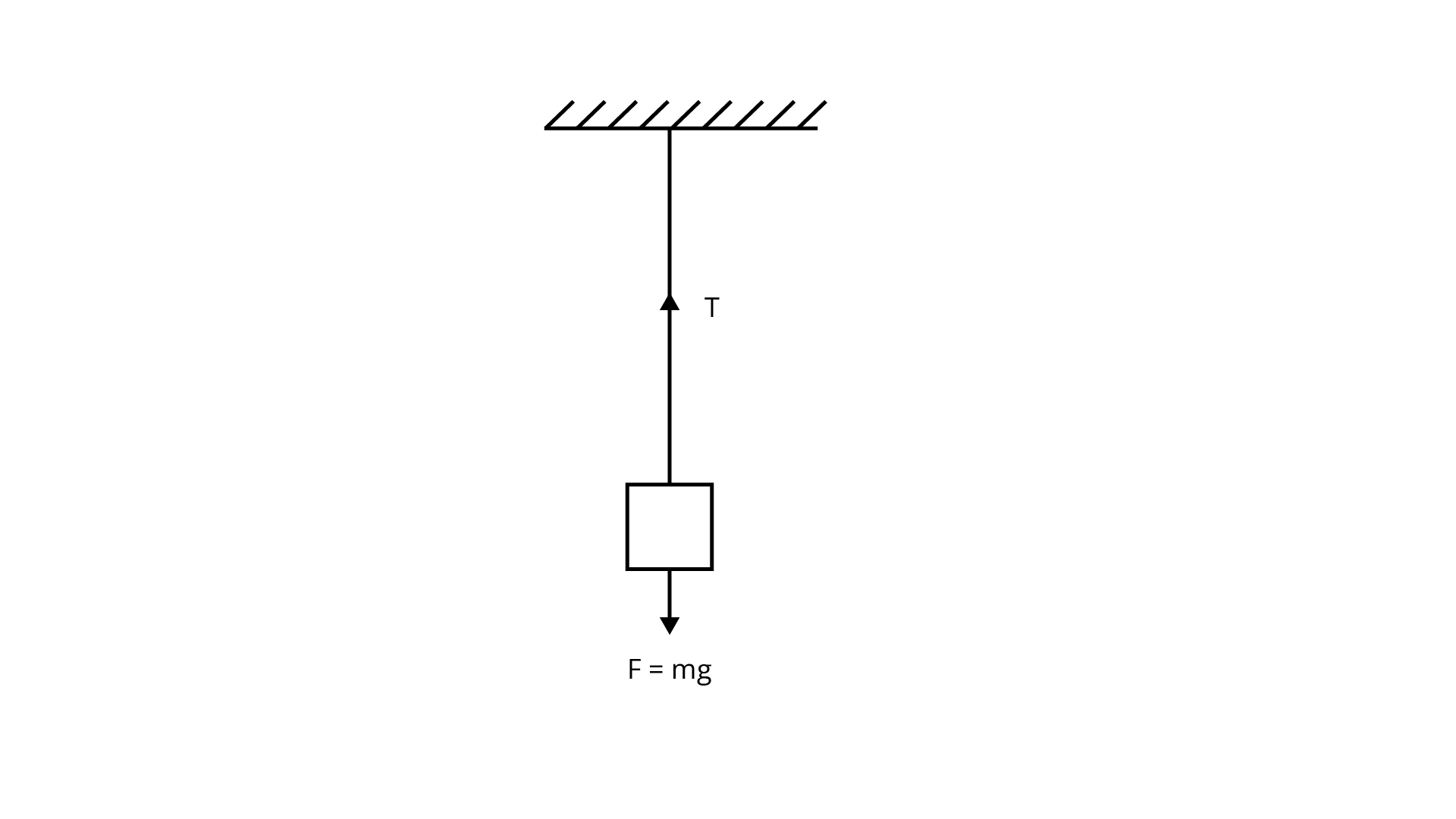(a) Tensile stress at any cross section ${\text{A}}$ of the wire is $\dfrac{{\text{F}}}{{\text{A}}}$

(b) Tensile stress at any cross section is zero.

(c) Tensile stress at any cross section ${\text{A}}$ of the wire is $\dfrac{{{\text{2F}}}}{{\text{A}}}$

(d) Tension at any cross section ${\text{A}}$ of the wire is ${\text{F}}$

Ans: Correct option is (a, d) because Stress ${\text{ = }}\dfrac{{\text{F}}}{{\text{A}}}$

So, tension is balanced by ${\text{F}}$

Thus, ${\text{T = F}}$

1. A rod of length 1 and negligible mass is suspended at its two ends by two wires of steel (wire A) and aluminium (wire B) of equal lengths (Figure). The cross-sectional areas of wires $\mathrm{A}$ and $\mathrm{B}$ are $1.0 \mathrm{mm}^ 2,2.0 \mathrm{mm}^2$ respectively.

$\left(\mathrm{Y}_{\mathrm{Al}}=70 \times 10^{9} \mathrm{~N} \times \mathrm{m}^{-2}, \mathrm{Y}_{\text {steel }}=200 \times 10^{9} \mathrm{Nm}^{-2}\right)$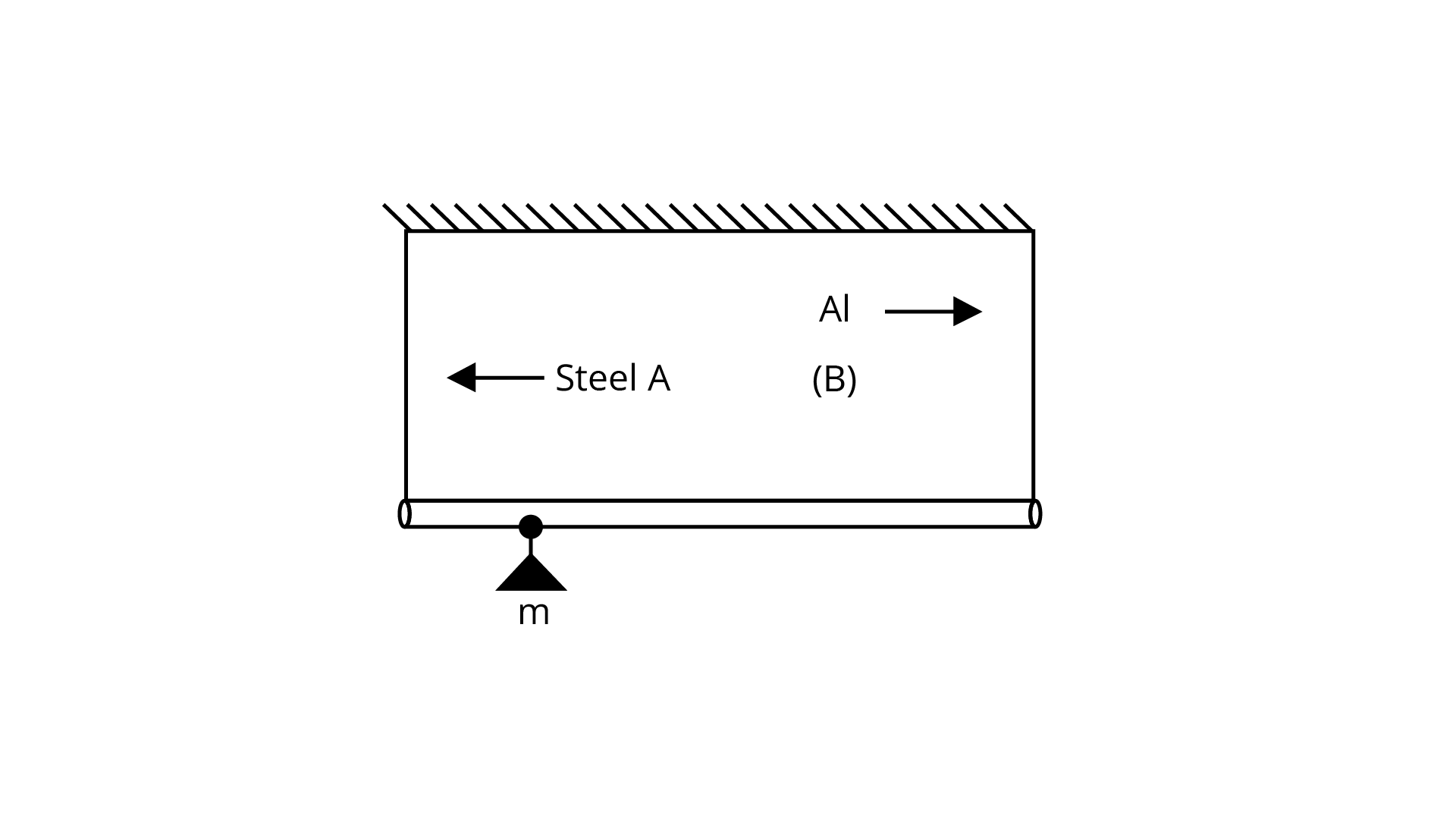(a) Mass ${\text{m}}$ should be suspended close to wire A to have equal stresses in both the wires.

(b) Mass ${\text{m}}$ should be suspended close to B to have equal stresses in both the wires.

(c) Mass ${\text{m}}$ should be suspended at the middle of the wires to have equal stresses in both the wires.

(d) Mass ${\text{m}}$ should be suspended close to wire A to have equal strain in both wires.

Ans: Correct option is (b, d) because

(b) Let mass ${\text{m}}$ at distance ${\text{x}}$ from wire B at C. Since rod is balanced by weight ${\text{mg}}$ at C. While taking moment about A, it is balanced by weight ${\text{mg}}$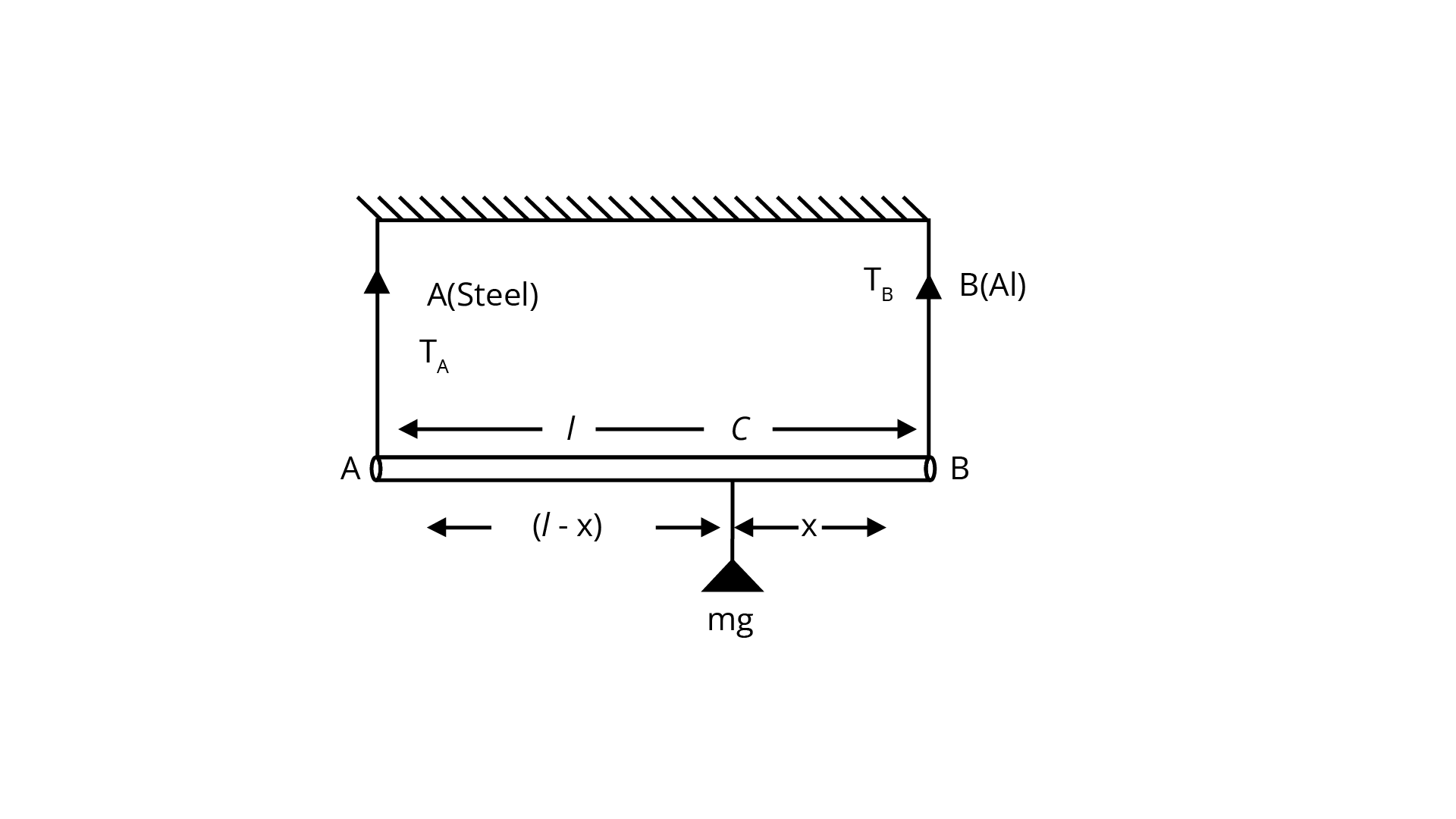$\mathrm{T}_{\mathrm{B}}(\mathrm{x})-\mathrm{T}_{\mathrm{A}}(1-\mathrm{x})=0$

$\mathrm{T}_{\mathrm{B}} \mathrm{x}=\mathrm{T}_{\mathrm{A}}(1-\mathrm{x})$

$\frac{\mathrm{T}_{\mathrm{B}}}{\mathrm{T}_{\mathrm{A}}}=\left(\frac{1-\mathrm{x}}{\mathrm{x}}\right)$

$\text { Stress in } \mathrm{A}=\frac{\mathrm{T}_{\mathrm{A}}}{\mathrm{A}_{\mathrm{A}}}$

Stress in $\mathrm{B}=\frac{\mathrm{T}_{\mathrm{B}}}{\mathrm{A}_{\mathrm{B}}}=\frac{\mathrm{T}_{\mathrm{B}}}{2 \mathrm{~A}_{\mathrm{A}}}$

$A_{\text {A }}=1 \mathrm{~mm}^{2}$

$A_{B}=2.0 \mathrm{~mm}^{2}$

$A_{B}=2 A_{\text {А }}$

Since stress on steel is equal to stress on aluminium, we get:

$\frac{T_{A}}{A_{A}}=\frac{T_{B}}{2 A_{A}}$

$T_{A}=\frac{T_{B}}{2}$

$\mathrm{T}_{\mathrm{B}}=2 \mathrm{~T}_{\mathrm{A}}$

${\mathrm{T}_{\mathrm{A}}}=2$

From above conditions we have

$\frac{1-x}{x}=\frac{2}{1}$

$2 x=1-x$

$3 x=1$

$x=\frac{1}{3}$ from B

Distance from $A=1-x=1-\frac{1}{3}=\frac{21}{3}$

Thus, mass is near to $B$ than $A$ wire

(d) As strain in both wires are equal because rod remains horizontal in balanced condition.

$(\text { strain })_{\mathrm{A}}=(\text { strain })_{\mathrm{B}}$

$\frac{\mathrm{S}_{\mathrm{A}}}{\mathrm{Y}_{\mathrm{A}}}=\frac{\mathrm{S}_{\mathrm{B}}}{\mathrm{Y}_{\mathrm{B}}}$

$\frac{\mathrm{Y}_{\mathrm{A}}}{\mathrm{S}_{\mathrm{A}}}=\frac{\mathrm{Y}_{\mathrm{B}}}{\mathrm{S}_{\mathrm{B}}} \quad\left[\because \mathrm{Y}=\frac{\text { stress }}{\text { strain }}\right]$

$\frac{\mathrm{Y}_{\text {stel }}}{\frac{\mathrm{T}_{\mathrm{A}}}{\mathrm{A}_{\mathrm{A}}}}=\frac{\mathrm{Y}_{\mathrm{A}}}{\mathrm{T}_{\mathrm{B}}}$

$\frac{\mathrm{Y}_{\mathrm{s} \text { stel }}}{\mathrm{Y}_{\mathrm{A}}}=\frac{\mathrm{T}_{\mathrm{A}}}{\mathrm{T}_{\mathrm{B}}} \times \frac{\mathrm{A}_{\mathrm{B}}}{\mathrm{A}_{\mathrm{A}}}=\left(\frac{\mathrm{x}}{1-\mathrm{x}}\right)\left(\frac{2 \mathrm{~A}_{\mathrm{A}}}{\mathrm{A}_{\mathrm{A}}}\right)$

$\frac{200 \times 10^{9}}{70 \times 10^{9}}=\frac{2 \mathrm{x}}{(\mathrm{l}-\mathrm{x})}$

$14 \mathrm{x}=201-20 \mathrm{x}$

$34 \mathrm{x}=20 \mathrm{I}$

$\mathrm{x}=\frac{20 \mathrm{I}}{34}=\frac{10 \mathrm{I}}{17} \text { from } \mathrm{B}$

$(\mathrm{l}-\mathrm{x}) \text { from } \mathrm{A}$

Thus, equal strain mass should be closer to $A$

1. For an ideal liquid

(a) the bulk modulus is infinite.

(b) the bulk modulus is zero.

(c) the shear modulus is infinite.

(d) the shear modulus is zero.

Ans: Correct option is (a, d) and rejects option (b, c) because as an ideal liquid is not compressible

Bulk modulus

${\text{(K) = }}\dfrac{{{\text{ - p(V)}}}}{{{\text{}\Delta{ V}}}}{\text{ (}}\because {\text{ }\Delta{ V = 0)}}$

As ${\text{}\Delta{ V = 0}}$ for ideal liquid

Therefore, ${\text{K = }}∞$ for ideal liquid

Since, no tangential force on liquid, so shearing strain

${\text{}\Delta{ θ = 0, F = 0}}$

${\text{Η = }}\dfrac{{\dfrac{{\text{F}}}{{\text{A}}}}}{{{\text{}\Delta{ θ}}}}{\text{ = }}\dfrac{{\text{0}}}{{\text{0}}}{\text{ = }}$

indeterminate value

1. A copper and a steel wire of the same diameter are connected end to end. A deforming force ${\text{F}}$ is applied to this composite wire which causes a total elongation of ${\text{1 cm}}$  The two wires will have: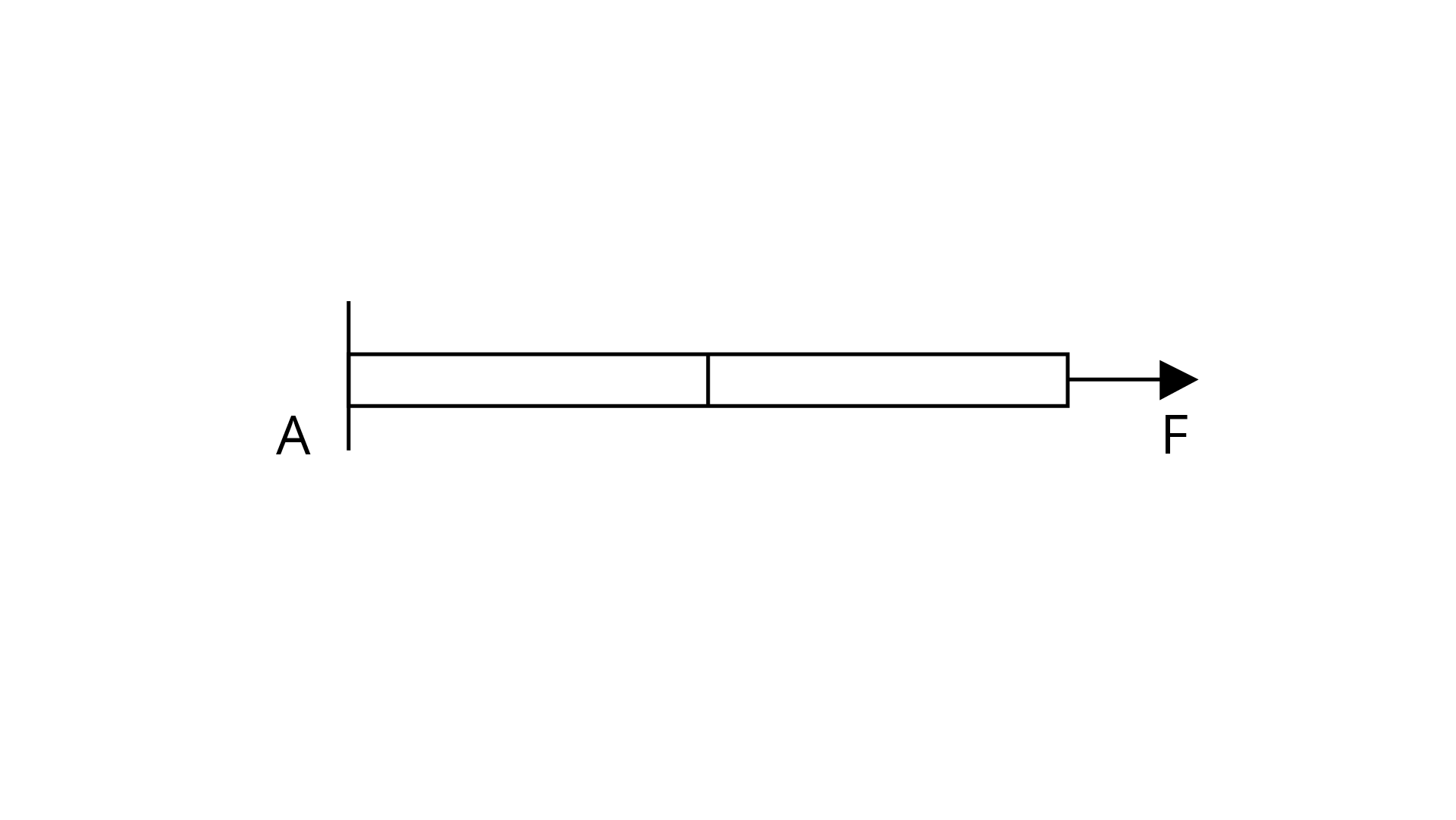(a) the same stress

(b) different stress

(c) the same strain

(d) different strain

Ans: Correct option is (a, d) because Stress ${\text{ = }}\dfrac{{\text{F}}}{{\text{A}}}$

As area of cross section for both wire is same and stretched by same force. Thus, stress of both are equal

Strain ${\text{ = }}\dfrac{{{\text{stress}}}}{{\text{Y}}}$

Since stress of both are equal then

${{\text{(Strain)}}_{{\text{steel}}}} \propto \dfrac{{\text{1}}}{{{{\text{Y}}_{{\text{steel }}}}}}{\text{, (Strain}}{{\text{)}}_{\text{A}}} \propto \dfrac{{\text{1}}}{{{{\text{Y}}_{\text{A}}}}}$

$\dfrac{{{{{\text{(Strain)}}}_{{\text{steel }}}}}}{{{{{\text{(Strain)}}}_{\text{A}}}}}{\text{ = }}\dfrac{{{{\text{Y}}_{\text{A}}}}}{{{{\text{Y}}_{{\text{zee }}}}}}$

${{\text{Y}}_{{\text{AL}}}}{\text{ < }}{{\text{Y}}_{\text{S}}}{\text{ }} \Rightarrow {\text{ }}\dfrac{{{\text{Al}}}}{{{\text{steel }}}}$

${{\text{(strain)}}_{{\text{steel }}}}{\text{ < (Strain}}{{\text{)}}_{\text{A}}}$

### Very Short Answer Type Questions

1. The Young's modulus for steel is much more than that for rubber. For the same longitudinal strain, which one will have greater tensile stress?

Ans: Here ${\text{Y = }}\dfrac{{{\text{ stress }}}}{{{\text{ strain }}}}$

According to question, longitudinal stress for rubber and steel are equal

So, ${\text{Y}} \propto {\text{Stress}}$

$\dfrac{{{{\text{Y}}_{{\text{Steel }}}}}}{{{{\text{Y}}_{{\text{Rubber }}}}}}{\text{ = }}\dfrac{{{{{\text{(Stress)}}}_{{\text{steel }}}}}}{{{{{\text{(Stress)}}}_{{\text{Rubber}}}}}}{\text{ }}\because {\text{ }}{{\text{Y}}_{{\text{steel }}}}{\text{ > }}{{\text{Y}}_{{\text{Rubber}}}}$

$\dfrac{{{{\text{Y}}_{{\text{steel}}}}}}{{{{\text{Y}}_{\text{A}}}}}{\text{ > 1}}$

${{\text{(Stress)}}_{_{{\text{steel}}}}}{\text{ > (Stress}}{{\text{)}}_{{\text{Rubber }}}}$

1. Is stress a vector quantity?

Ans: Stress ${\text{ = }}\dfrac{{{\text{ Magnitude of restoring force by solid }}}}{{{\text{ Area of cross section }}}}$

Since deforming and restoring force are equal and opposite. Thus, net direction is included.

So, stress is not a vector quantity which is just like pressure.

1. Identical springs of steel and copper are equally stretched. On which, more work will have to be done?

Ans: As two identical springs are equally stretched by same force.

So, Spring I (steel)

$F_{1}=F$

$Y_{1}=Y_{\mathrm{s}}$

$L_{1}=L$

$A_{1}=A$

$\Delta L=\Delta L_{1} \quad \because Y=\frac{F L}{A \Delta L}$

For spring II (copper)

$\mathrm{F}_{2}=\mathrm{F}$

$\mathrm{Y}_{2}=\mathrm{Y}_{\mathrm{cu}}$

$\mathrm{L}_{2}=\mathrm{L}$

$\mathrm{A}_{2}=\mathrm{A}$

$\Delta \mathrm{L}^{\prime}=\Delta \mathrm{L}_{2} \quad \because \Delta \mathrm{L}=\frac{\mathrm{FL}}{\mathrm{AY}}$

Now, wires

$\Delta \mathrm{L} \propto \frac{1}{\mathrm{Y}}$

$\frac{\Delta \mathrm{L}_{1}}{\Delta \mathrm{L}_{2}}=\frac{\mathrm{Y}_{\mathrm{c}}}{\mathrm{Y}_{\mathrm{s}}} \quad\left[\because \mathrm{WD}=\mathrm{F} \times \Delta \mathrm{L}, \mathrm{F}_{1}=\mathrm{F}_{2}=\mathrm{F}, \mathrm{WD} \propto \Delta \mathrm{L}\right]$

$\frac{\mathrm{WD}_{\mathrm{s}}}{\mathrm{WD}_{\mathrm{c}}}=\frac{\mathrm{Y}_{\mathrm{c}}}{\mathrm{Y}_{\mathrm{s}}} \quad\left(\because \mathrm{Y}_{\mathrm{s}}>\mathrm{Y}_{\mathrm{c}}\right)$

$\frac{\mathrm{Y}_{\mathrm{c}}}{\mathrm{Y}_{\mathrm{s}}}<1$

$\frac{\mathrm{WD}_{\mathrm{s}}}{\mathrm{WD}_{\mathrm{c}}}<1 \mathrm{WD}_{\mathrm{s}}<\mathrm{WD}_{\mathrm{c}}$

Thus, work done is more stretch the copper string.

1. What is the Young’s modulus for a perfect rigid body?

Ans: ${\text{Y = }}\dfrac{{{\text{FL}}}}{{{\text{A}\Delta{ L}}}}$ is a rigid body which cannot be de-shaped by applying any deforming force

Therefore, ${\text{}\Delta{ L = 0}}$

${\text{Y = }}\dfrac{{{\text{FL}}}}{{{\text{A(0)}}}}{\text{ = }}\infty$ which is a perfect rigid body. Hence Young’s modulus for a perfect rigid body is infinity.

1. What is the Bulk modulus for a perfect rigid body?

Ans: Bulk Modulus ${\text{ = }}\dfrac{{{\text{ - p(V)}}}}{{{\text{}\Delta{ V}}}}$ since perfect rigid body doesn’t change it's shape even at infinite  force.

Thus, ${\text{}\Delta{ V = 0}}$

which implies ${\text{B = }}\dfrac{{{\text{pV}}}}{{{\text{}\Delta{ V}}}}{\text{ = }}\dfrac{{{\text{pV}}}}{{\text{0}}}{\text{ = }}\infty$

Hence, bulk modulus is infinity.

1. A wire of length ${\text{L}}$ and radius ${\text{r}}$ is clamped rigidly at one end. When the other end of the wire is pulled by a force ${\text{f}}$ , its length increases by ${\text{l}}$ . Another wire of the same material of length ${\text{2L}}$ and radius ${\text{2r}}$ , is pulled by a force ${\text{2f}}$ . Find the increase in length of this wire.

Ans: Ans:

Wire 1

$\mathrm{L}_{1}=\mathrm{L}$

$\mathrm{f}_{1}=\mathrm{r}$

$\mathrm{A}_{1}=\pi \mathrm{r}^{2}$

$\mathrm{~F}_{1}=\mathrm{f}$

$\Delta \mathrm{L}_{1}=1$

$\mathrm{Y}_{1}=\mathrm{Y}$

Wire 2

$\mathrm{L}_{2}=2 \mathrm{~L}$

$\mathrm{f}_{2}=2 \mathrm{r}$

$\mathrm{A}_{2}=\pi(2 \mathrm{r})^{2}=4 \pi \mathrm{r}^{2}$

$\mathrm{~F}_{2}=2 \mathrm{f}$

$\Delta \mathrm{L}_{2}=?$

$\mathrm{Y}_{2}=\mathrm{Y}$

$\mathrm{As} \mathrm{Y}=\frac{\mathrm{FL}}{\mathrm{A} \Delta \mathrm{L}} \text { or } \Delta \mathrm{L}=\frac{\mathrm{FL}}{\mathrm{AY}}$

$\frac{\mathrm{F}_{2} \mathrm{~L}_{2}}{\mathrm{~A}_{2} \mathrm{Y}_{2}}=\frac{\mathrm{F}_{2} \mathrm{~L}_{2}}{\mathrm{~F}_{1} \mathrm{~L}_{1}} \times \frac{\mathrm{A}_{1} \mathrm{Y}_{1}}{\mathrm{~A}_{2} \mathrm{Y}_{2}}=\frac{2 \mathrm{f} 2 \mathrm{~L}}{\mathrm{fL}} \times \frac{\pi \mathrm{r}^{2} \times \mathrm{Y}}{4 \pi \mathrm{r}^{2} \times \mathrm{Y}}$

$\frac{\mathrm{F}_{1} \mathrm{~L}_{1}}{\mathrm{~A}_{1} \mathrm{Y}_{1}}$

$\frac{\Delta \mathrm{L}_{2}}{1}=\frac{4}{4}=1$

$\Delta \mathrm{L}_{2}=1$

Thus, change in length of second wire is also same that is one.

1. A steel rod $\left( {{\text{Y = 2 }\times{ 1}}{{\text{0}}^{{\text{11}}}}{\text{N}}{{\text{m}}^{{\text{ - 2}}}}} \right.{\text{ and }\alpha{ = 10 - 5}}{{\text{c}}^{{\text{ - 1}}}}{\text{)}}$ of length ${\text{1m}}$ and area of cross-section ${\text{1 c}}{{\text{m}}^2}$ is heated from ${{\text{0}}^\circ}{\text{C to 20}}{{\text{0}}^\circ}{\text{C}}$ , without being allowed to extend or bend. What is the tension produced in the rod?

Ans: ${{\text{L}}_{\text{t}}}{\text{ = }}{{\text{L}}_{\text{0}}}{\text{(1 + α}\Delta{t)}}$

${{\text{L}}_{\text{t}}}{\text{ - }}{{\text{L}}_{\text{0}}}{\text{ = }}{{\text{L}}_{\text{0}}}{\text{α ×}\Delta{ t}}$

${\text{}\Delta{ L = 1 × 1}}{{\text{0}}^{{\text{ - 5}}}}{\text{ × 200 = 2 ×1}}{{\text{0}}^{{\text{ - 3}}}}$

${\text{Y = }}\dfrac{{{\text{F}}{{\text{L}}_{\text{0}}}}}{{{\text{A}\Delta{L}}}}{\text{ }}$

${{\text{L}}_{\text{0}}}{\text{ = 1m}}$

${\text{F = }}\dfrac{{{\text{YA}\Delta{ L}}}}{{{{\text{L}}_{\text{0}}}}}$

${\text{A = 1c}}{{\text{m}}^{\text{2}}}{\text{ = 1}}{{\text{0}}^{{\text{ - 4}}}}{\text{}}{{\text{m}}^{\text{2}}}$

${\text{Y = 2 × 1}}{{\text{0}}^{{\text{11}}}}{\text{N}}{{\text{m}}^{{\text{ - 2}}}}$

${\text{}\Delta{ L = 2 × 1}}{{\text{0}}^{{\text{ - 3}}}}{\text{m}}$

${\text{F = }}\dfrac{{{\text{2 ×1}}{{\text{0}}^{{\text{11}}}}{\text{ × 1}}{{\text{0}}^{{\text{ - 4}}}}{\text{ × 2 × 1}}{{\text{0}}^{{\text{ - 3}}}}}}{{\text{1}}}$

${\text{ = 4 × 1}}{{\text{0}}^{{\text{11 - 7}}}}{\text{ = 4 × 1}}{{\text{0}}^{\text{4}}}{\text{N}}$

1. To what depth must a rubber ball be taken in deep sea so that its volume is decreased by ${\text{0}}{\text{.1% }}$ . The bulk modulus of rubber is ${\text{9}}{\text{.8 }\times{ 1}}{{\text{0}}^{\text{8}}}{\text{N}}{{\text{m}}^{{\text{ - 2}}}}$ ; and the density of sea water is ${\text{1}}{{\text{0}}^{\text{3}}}{\text{kg}}{{\text{m}}^{{\text{ - 3}}}}$

Ans: Bulk modulus ${\text{ = B = 9}}{\text{.8 × 1}}{{\text{0}}^{\text{8}}}{\text{ N}}{\text{.}}{{\text{m}}^{{\text{ - 2}}}}$

Density of water ${\text{ = ρ = 1}}{{\text{0}}^{\text{3}}}{\text{kg}}{{\text{m}}^{{\text{ - 3}}}}$

Percentage change in volume

${\text{ = }}\dfrac{{{\text{}\Delta{ V}}}}{{\text{V}}}{\text{ × 100 = 0}}{\text{.1}}$

$\dfrac{{{\text{}\Delta{ V}}}}{{\text{V}}}{\text{ = }}\dfrac{{\text{1}}}{{{\text{1000}}}}$

Let us consider rubber ball upto depth ${\text{h}}$ in sea

${\text{p = hρ g = h × 1}}{{\text{0}}^{\text{3}}}{\text{ × 9}}{\text{.8N}}{{\text{m}}^{\text{2}}}$

${\text{B = }}\left| {\dfrac{{{\text{ - p}}}}{{{\text{}\Delta{ V}}}}} \right|{\text{ or p = B }\times{ }}\dfrac{{{\text{}\Delta{ V}}}}{{\text{V}}}$

${\text{h × 9}}{\text{.8 × 1}}{{\text{0}}^{\text{3}}}{\text{ = 9}}{\text{.8 × 1}}{{\text{0}}^{\text{8}}}{\text{ × }}\dfrac{{\text{1}}}{{{\text{1000}}}}$

${\text{h = }}\dfrac{{{\text{9}}{\text{.8 × 1}}{{\text{0}}^{\text{8}}}}}{{{\text{1000 × 9}}{\text{.9 × 1}}{{\text{0}}^{\text{3}}}}}{\text{ = 1}}{{\text{0}}^{{\text{ + 8 - 6}}}}{\text{ = 1}}{{\text{0}}^{\text{2}}}$

${\text{h = 100 m}}$

Hence, ball is ${\text{100 m}}$ below sea.

1. A truck is pulling a car out of a ditch by means of a steel cable that is ${\text{9}}{\text{.1m}}$ long and has a radius of ${\text{5mm}}$ . When the car just begins to move, the tension in the cable is ${\text{800N}}$ . How much has the cable stretched? (Young's modulus for steel is ${\text{2 }\times{ 1}}{{\text{0}}^{{\text{11}}}}{\text{N }\times{ }}{{\text{m}}^{{\text{ - 2}}}}$)

Ans: Let length of cable denoted as $\mathrm{L}$ which is given as $\mathrm{L}=9.1 \mathrm{~m}$

$\mathrm{r}=5 \mathrm{~mm}=5 \times 10^{-3} \mathrm{~m}$

$\mathrm{~A}=\pi \mathrm{r}^{2}$

Tension in cable $=\mathrm{F}=800 \mathrm{~N}$

$\mathrm{Y}=2 \times 10^{11} \mathrm{Nm}^{-2}$

$\Delta \mathrm{L}=\frac{\mathrm{FL}}{\mathrm{AY}}=\frac{800 \times 9.10}{3.14 \times 10^{-3} \times 10^{-3} \times 5 \times 5 \times 2 \times 10^{11}}$

$\Delta \mathrm{L}=\frac{800 \times 910 \times 10^{-11+6}}{314 \times 5 \times 5 \times 2}$

$=\frac{728}{157} \times 10^{-5} \mathrm{~m}=4.64 \times 10^{-5} \mathrm{~m}$

$\Delta \mathrm{L}=4.64 \times 10^{-5} \mathrm{~m}$

1. Two identical solid balls, one of ivory and the other of wet-clay, are dropped from the same height on the floor. Which one will rise to a greater height after striking the floor and why?

Ans: As the ivory ball is more elastic than wet clay ball and both balls are dropped from same height.

So, velocity of both ball just before striking the floor will be same. Thus, ivory ball tries to regain its original shape quickly and tries to change shape which is negligible for ivory ball as compared to wet clay ball. Finally, more energy is transferred to ivory ball as compared to wet clay ball. Hence, ivory ball rises more as compared to clay ball.

1. Consider a long steel bar under a tensile stress due to forces acting at the edges along the length of the bar (Figure). Consider a plane making an angle ${\text{}\theta{ }}$ with the length. What are the tensile and shearing stresses on this plane?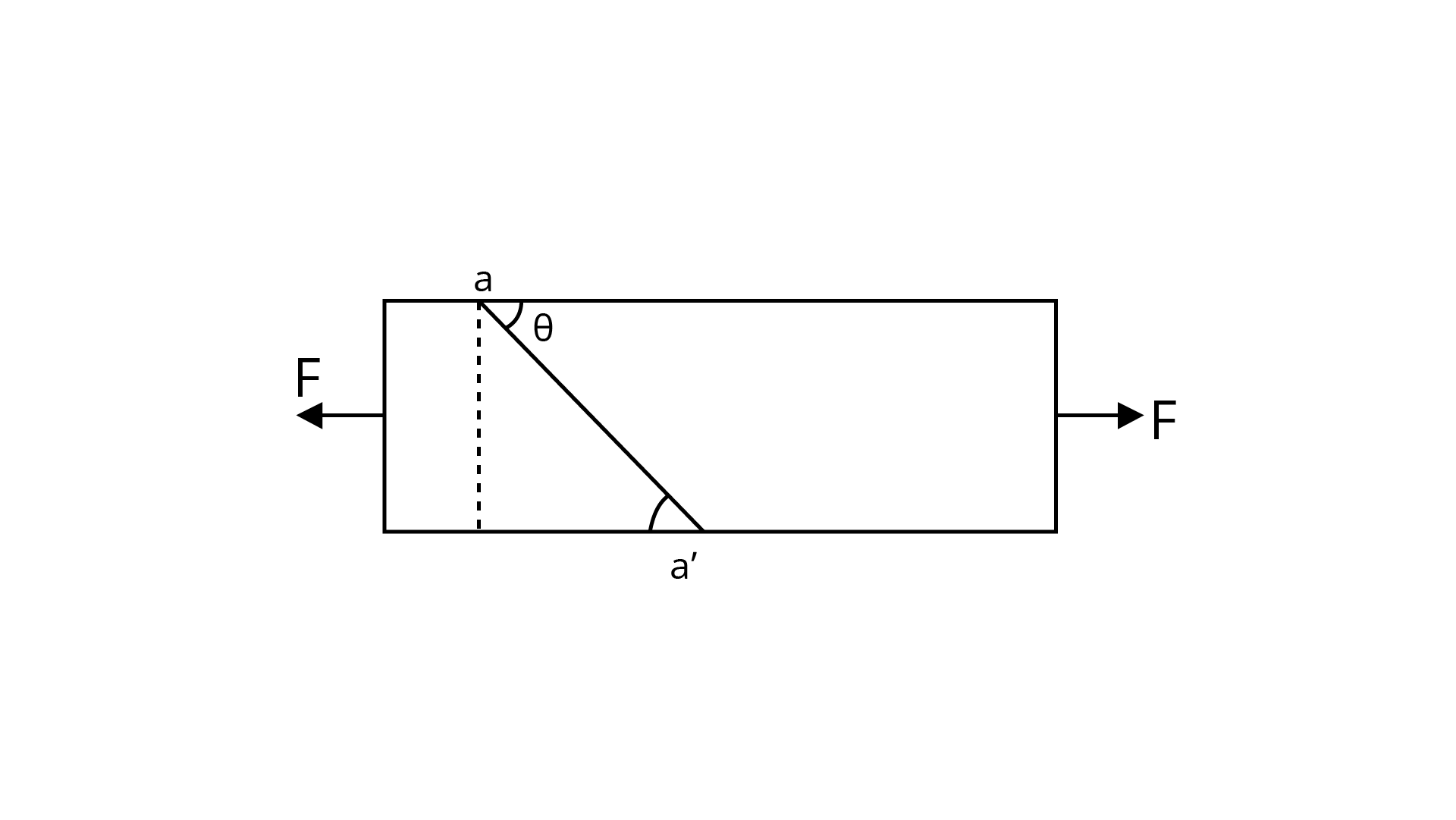(a) For what angle is the tensile stress a maximum?

Ans: Tensile stress $=\frac{\text { Forces normal to surface }\left(F_{p}\right)}{\text { Area of surface }}$

Let A represents area of cross-section which is perpendicular to the length of bar

And A represents area of cross-section plane which cut along aa$\sin \theta=\frac{\mathrm{A}}{\mathrm{A}^{\prime}}$

$\mathrm{A}^{\prime}=\frac{\mathrm{A}}{\sin \theta}$

So component of force which is perpendicular to plane $A^{\prime}$ or $a a^{\prime}=F \sin \theta=F_{p}$

Therefore, tensile stress $=\frac{F \sin \theta}{A} \times \sin \theta=\frac{F}{A} \sin ^{2} \theta$

Now, for maximum tensile stress $\sin ^{2} \theta=1$

$\sin \theta=1$

$\sin \theta=\sin 90^{\circ}$

$\theta=\frac{\pi}{2}$

(b) For what angle is the shearing stress a maximum?

Ans: shearing stress =$\frac{\text { Force along the plane } F_{p}}{\text { Area of plane }}$

$=\frac{F \cos \theta}{A^{\prime}}=\frac{F \cos \theta \sin \theta}{A}=\frac{F}{2 A} \times 2 \sin \theta \cos \theta$

$\text { Shearing stress }=\frac{F}{2 A} \sin 2 \theta$

Now, for maximum shearing stress

$\sin 2 \theta=1$

$\sin 2 \theta=\sin 90^{\circ}$

$2 \theta=90^{\circ}$

$\theta=\frac{90^{\circ}}{2}=45^{\circ}=\frac{\pi}{4}$

1. (a) A steel wire of mass $\mu$ per unit length with a circular cross section has a radius of $0.1 \mathrm{~cm}$. The wire is of length $10 \mathrm{~m}$ when measured lying horizontal, and hangs from a hook on the wall. A mass of $25 \mathrm{~kg}$ is hung from the free end of the wire. Assuming the wire to be uniform and lateral strains $<<$ longitudinal strains, find the extension in the length of the wire. The density of steel is $7860 \mathrm{~kg} \mathrm{~m}^{-3}$ (Young's modules $\mathrm{Y}=2 \times 10^{11} \mathrm{~N} \times \mathrm{m}^{-2}$ )

Ans: Let small element be $\mathrm{dx}$ with mass $\mathrm{dm}$ from length $\mathrm{L}$ at distance $\mathrm{x}$ from the end of hung weight. $\mu$ represents mass per unit length

$\mathrm{dm}=\mu . \mathrm{dx}$

$\mathrm{r}=0.1 \mathrm{~cm}=0.1 \times 10^{-2} \mathrm{~m}=10^{-3} \mathrm{~m}$

$\mathrm{A}=\pi \mathrm{r}^{2}=\pi(10)^{-6}$

$\mathrm{M}=25 \mathrm{~kg}$

$\mathrm{~L}=10 \mathrm{~m}$

So, downward force on $\mathrm{dx}=\omega \mathrm{t}$ below $(\mathrm{dx})$

$\mathrm{T}(\mathrm{x})=(\mathrm{x} \times \mu) \mathrm{g}+\mathrm{Mg}$

$\mathrm{Y}=\frac{\frac{\mathrm{T}(\mathrm{x})}{\mathrm{A}}}{\frac{\mathrm{dr}}{\mathrm{dx}}}$

When dr increases in length of wire by $\mathrm{T}(\mathrm{x})$

$\mathrm{Y} =\frac{\mathrm{T}(\mathrm{x}) \times \mathrm{dx}}{\mathrm{A} \times \mathrm{dr}}$

$\frac{\mathrm{dr}}{\mathrm{dx}}=\frac{\mathrm{T}(\mathrm{x})}{\mathrm{AY}}$

$\mathrm{dr}=\frac{1}{\mathrm{AY}}(\mathrm{x} \mu \mathrm{g}+\mathrm{Mg}) \mathrm{dx}$

Now, integrating both sides we get:

$\int_{0}^{\mathrm{r}} \mathrm{dr}=\frac{1}{\mathrm{AY}} \int_{0}^{\mathrm{L}}(\mathrm{x} \mu \mathrm{g}+\mathrm{Mg}) \mathrm{dx}$

$\mathrm{r}=\frac{1}{\mathrm{AY}}\left[\mu \mathrm{g} \frac{\mathrm{x}^{2}}{2}+\mathrm{Mgx}\right]_{0}^{\mathrm{L}}$

Extension in wire of length $\mathrm{L}=\frac{\mathrm{gL}}{2 \pi \mathrm{r}^{2} \mathrm{Y}}[\mu \mathrm{L}+2 \mathrm{M}] \quad \because \mu \mathrm{L}=\mathrm{m}$

Therefore, extension $=\frac{\mathrm{gl}}{2 \mathrm{AY}} \quad[\mathrm{m}=2 \mathrm{~m}]$

$=\frac{10 \times 10[0.25+2 \times 25]}{2 \times 3.14 \times 10^{-6} \times 2 \times 10^{11}}=\frac{100[.25+50]}{2 \times 6.28 \times 10^{5}}=\frac{100 \times 5025 \times 10^{-5}}{2 \times 628}$

$=\frac{502500}{2 \times 628} \times 10^{-5}=400 \times 10^{-5} \mathrm{~m}$

$\mathrm{~m}=$ vol $\times$ density

$=(\mathrm{A} . \mathrm{L}) \times \mathrm{P}$

$=\pi \mathrm{r}^{2} \times 10 \times 7860$

$=3.14 \times(7860) \times\left(10^{-3}\right)^{2} \times 10$

$=3.14 \times(7860) \times 10^{-6} \times 10$

$=2468.04 \times 10^{-4} \mathrm{~kg}$

$\mathrm{~m} \cong 0.25 \mathrm{~kg}$

(b) If the yield strength of steel is $2.5 \times 10^{8} \mathrm{~N} \times \mathrm{m}^{-2}$, what is the maximum weight that can be hung at the lower end of the wire?

Ans: Here let tension in wire will be maximum at $x=L$

$\mathrm{T}(\mathrm{x})=\mu \mathrm{gx}+\mathrm{Mg}$

$\mathrm{T}(\mathrm{L})=\mu \mathrm{g} \mathrm{L}+\mathrm{Mg}$

$\mathrm{T}=\mu \mathrm{Ig}+\mathrm{Mg} \quad(\because \mu \mathrm{L}=\mathrm{m})$

$\mathrm{T}=(\mathrm{m}+\mathrm{M} \mathrm{g}$

Yield force $=[$ Yield strength $(\mathrm{Y})] \times \mathrm{A}$

$=2.5 \times 10^{8} \times 3.14 \times 10^{6}$

Yield force $=250 \times 3.14$

Yield force $=\mathrm{tension}($ which is maximum $)$

$250 \times 3.14=(\mathrm{m}+\mathrm{M}) \mathrm{g}$

$\frac{250 \times 3.14}{\mathrm{~g}}=[0.25+\mathrm{M}]$

$\frac{785}{10}-0.25=\mathrm{M}$

$\mathrm{M}=78.5-0.25$

$\mathrm{M}=78.25 \mathrm{Kg}$

1. A steel rod of length ${\text{2 l}}$ , cross sectional area ${\text{A}}$ and mass ${\text{M}}$ is set rotating in a horizontal plane about an axis passing through the centre. If ${\text{Y}}$ is the Young's modulus for steel, find the extension in the length of the rod. (Assume the rod is uniform.)

Ans: From figure an element ${\text{dr}}$ at distance ${\text{r}}$ from centre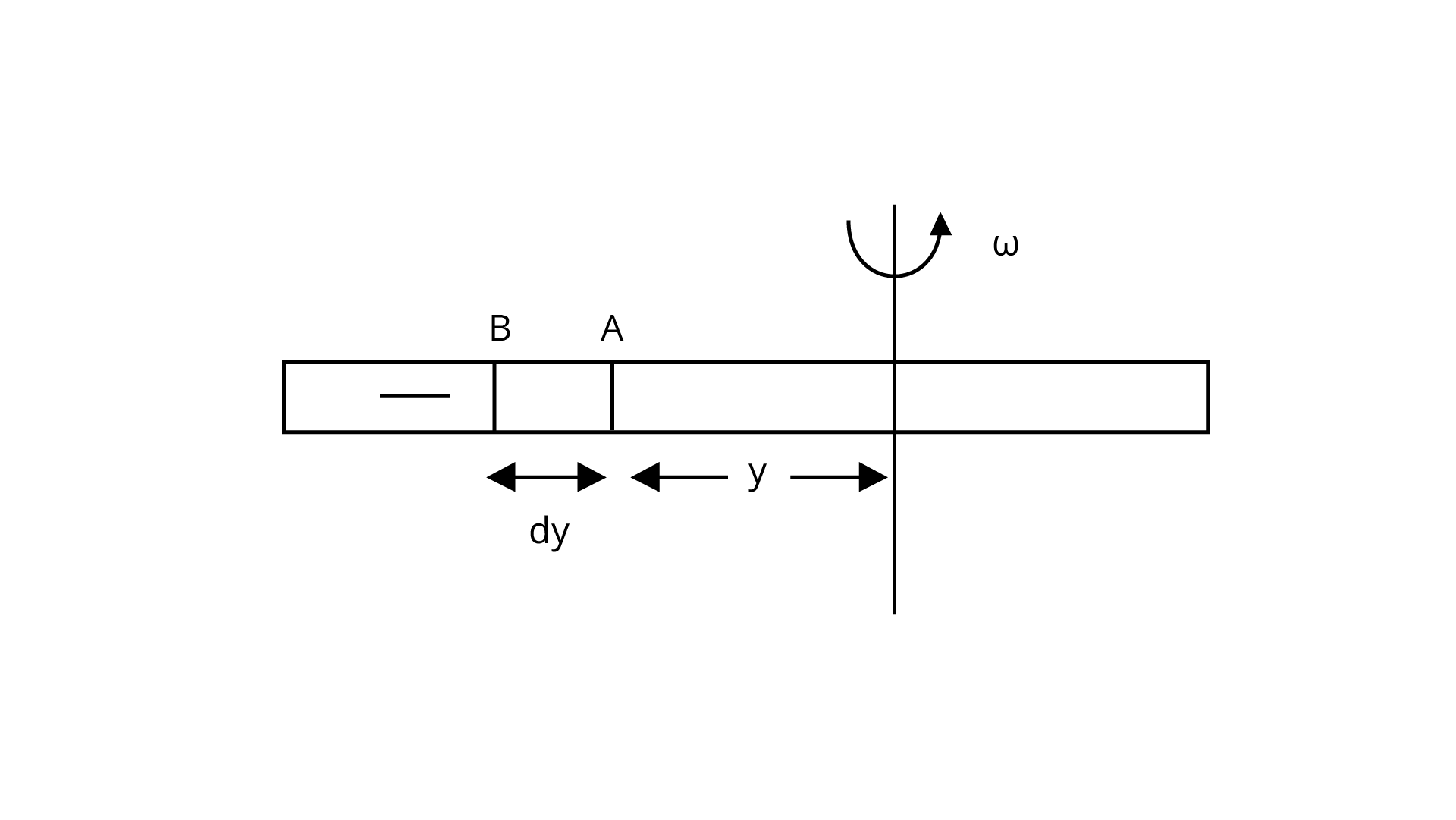Assume $\mathrm{T}(\mathrm{r}), \mathrm{T}(\mathrm{r}+\mathrm{dr})$ are the tensions external force to rod extend at $\mathrm{A}, \mathrm{B}$ ends respectively.

So, centrifugal force on dr due to tension difference $=\mathrm{T}(\mathrm{r}+\mathrm{dr})-\mathrm{T}(\mathrm{r})$

Centrifugal force $=-\mathrm{dT}$ (outward)

Centrifugal force due to rotation $\mathrm{dr}=\mathrm{dm} \mathrm{rw}^{2}$

Therefore, $-\mathrm{dt}=\mathrm{dm} \omega^{2} \mathrm{r}$

Now, let mass per unit length be $\mu$

Then $-\mathrm{dT}=\omega^{2} \mathrm{r}(\mathrm{dr} \cdot \mu)$

$-\mathrm{dT}=\mu \mathrm{w}^{2} \mathrm{r} \times \mathrm{dr}$

Now, integrate on both sides:

$-\int_{0}^{T} \mathrm{dT}=\mu \mathrm{w}^{2} \int_{\mathrm{r}}^{1} \mathrm{r} \mathrm{dr}$

So, tension in rod at $r$ from centre and here limits vary from $r$ to 1

$-\mathrm{T}(\mathrm{r})=\mu \omega^{2}\left[\frac{\mathrm{r}^{2}}{2}\right]_{\mathrm{r}}^{1}=\frac{\mu \omega^{2}}{2}\left(1^{2}-\mathrm{r}^{2}\right)$

(i) Further, let increase in length of $\mathrm{dr}$ at $\mathrm{r}$ from centre is $\delta \mathrm{r}$, then we have

$\mathrm{Y}=\frac{\text { Strtess }}{\text { Strain }}=\frac{\frac{\mathrm{T}(\mathrm{r})}{\mathrm{A}}}{\frac{\delta \mathrm{r}}{\mathrm{dr}}}=\frac{\mathrm{T}(\mathrm{r})}{\mathrm{A}} \times \frac{\mathrm{dr}}{\delta \mathrm{r}}$

$\frac{\delta \mathrm{r}}{\mathrm{dr}}=\frac{\mathrm{T}(\mathrm{r})}{\mathrm{AY}}=\frac{-\mu \mathrm{w}^{2}}{2 \mathrm{AY}}\left(1^{2}-\mathrm{r}^{2}\right)$

Since negative sign shows only direction of extension which is opposite to restoring force

$\delta \mathrm{r}=\frac{\mu \mathrm{w}^{2}}{2 \mathrm{AY}}\left(1^{2}-\mathrm{r}^{2}\right) \mathrm{dr}$

$\int_{0}^{5} \delta \mathrm{r}=\int_{0}^{1} \frac{\mu \mathrm{w}^{2}}{2 \mathrm{AY}}\left(1^{2}-\mathrm{r}^{2}\right) \mathrm{dr}$

$\delta=\frac{\mu \mathrm{w}^{2}}{2 \mathrm{AY}}\left(1^{3}-\frac{1^{3}}{3}\right)=\frac{\mu \mathrm{w}^{2}}{2 \mathrm{AY}} \frac{2}{3} 1^{3}$

$\delta=\frac{\mu \mathrm{w}^{2}}{3 \mathrm{AY}} 1^{3}$

Therefore, total extension in rod both sides is $2 \delta=\frac{2 \mu \mathrm{w}^{2} \mathrm{l}^{3}}{3 \mathrm{AY}}$

1. An equilateral triangle $A B C$ is formed by two $\mathrm{Cu}$ rods $A B$ and $B C$ and one $Al$ rod. It is heated in such a way that temperature of each rod increases $\Delta \mathrm{T}$. Find change in the angle $A B C$. Coefficient of linear expansion for Cu is $\alpha_{1}$, Coefficient of linear expansion for Al is $\alpha_{2}$.

Ans: We can solve this by using trigonometry

$\cos \theta=\frac{1_{1}^{2}+l_{3}^{2}-l_{2}^{2}}{2 l_{1} l_{3}}$

$2 l_{1} 1_{3} \cos \theta=1_{1}^{2}+l_{3}^{2}-l_{2}^{2}$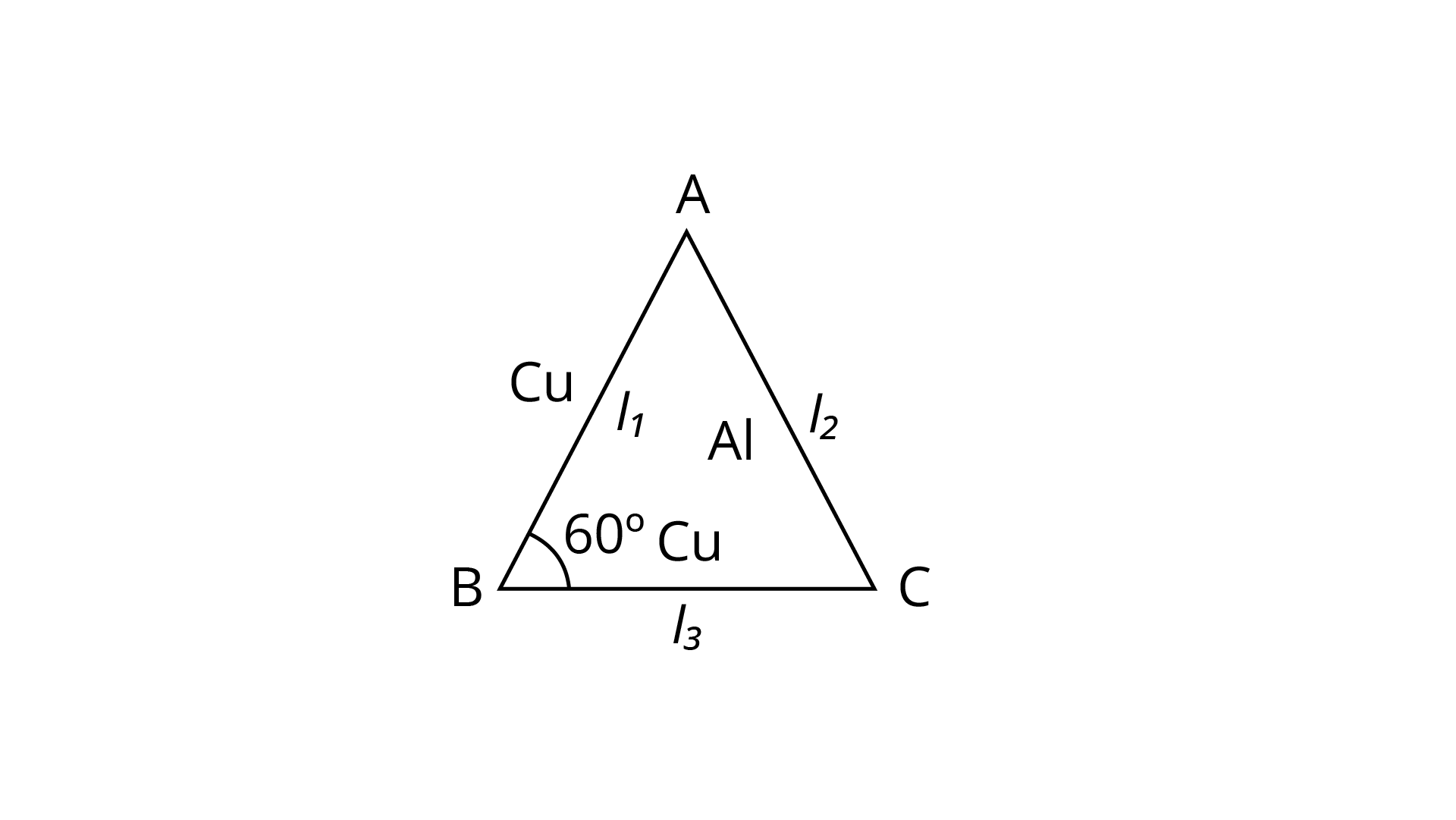Now, differentiating both sides:

$2\left[\mathrm{~d}\left(1_{1} 1_{3}\right) \times \cos \theta+\mathrm{l}_{1} \mathrm{l}_{3} \mathrm{~d}(\cos \theta)\right]=2 \mathrm{l}_{1} \mathrm{dl}_{1}+2 \mathrm{l}_{3} \mathrm{dl}_{3}-2 \mathrm{l}_{2} \mathrm{dl}_{2}$

$2\left[\left(1_{1} \mathrm{dl}_{3}+1_{3} \mathrm{dl}_{1}\right) \cos \theta-1_{1} 1_{3} \sin \theta \mathrm{d} \theta\right]=2\left(1_{1} \mathrm{dl}_{1}+\mathrm{l}_{3} \mathrm{dl}_{3}-1_{2} \mathrm{dl}_{2}\right)$

$\left(1_{1} \mathrm{dl}_{3}+1_{3} \mathrm{dl}_{1}\right) \cos \theta-1_{1} 1_{3} \sin \theta \mathrm{d} \theta=1_{1} \mathrm{dl}_{1}+1_{3} \mathrm{dl}_{3}-1_{2} \mathrm{dl}_{2}$

$\mathrm{~L}_{\mathrm{t}}=\mathrm{L}_{0}(1+\alpha \Delta \mathrm{t})$

$\mathrm{L}_{\mathrm{t}}-\mathrm{L}_{0}=\mathrm{L}_{0} \alpha \Delta \mathrm{t}$

$\Delta \mathrm{L}=\mathrm{L} \alpha \times \Delta \mathrm{t}$

$\mathrm{dl}_{1}=1_{1} \alpha_{1} \Delta \mathrm{t}, \mathrm{dl}_{3}=1_{2} \alpha_{1} \Delta \mathrm{t}$

$\mathrm{dl}_{2}=1_{2} \alpha_{2} \Delta \mathrm{t}$

$1_{1}=1_{2}=1_{3}=1$

$\mathrm{dl}_{1}=1 \mathrm{l}_{1} \Delta \mathrm{t}, \mathrm{dl}_{3}=1 \alpha_{1} \Delta \mathrm{t}$

$\mathrm{dl}_{2}=1 \alpha_{2} \Delta \mathrm{t}$ (i)

Now, substitute in (i)

$\cos \theta\left(1^{2} \times \alpha_{1} \Delta t+1^{2} \alpha_{1} \Delta t\right)-1^{2} \sin \theta d \theta=l^{2} \alpha_{1} \Delta t+l^{2} \alpha_{1} \Delta t-1^{2} \alpha_{2} \Delta t$

$2 l^{2} \alpha_{1} \Delta t \cos \theta-l^{2}[\sin \theta \times d \theta]=1^{2}\left[\alpha_{1}+\alpha_{1}-\alpha_{2}\right] \Delta t$

$1^{2}\left[2 \alpha_{1} \Delta \cos 60^{\circ}-\sin 60^{\circ} \mathrm{d} \theta\right]=1^{2}\left[2 \alpha_{1}-\alpha_{2}\right] \Delta t$

$2 \alpha_{1} \Delta t \times \frac{1}{2}-2 \alpha_{1} \Delta t+\alpha_{2} \Delta t=\frac{\sqrt{3}}{2} \mathrm{~d} \theta$

$\frac{\sqrt{3}}{2} \mathrm{~d} \theta=\left[\alpha_{1}-2 \alpha_{1}+\alpha_{2}\right] \Delta \mathrm{t}$

$\mathrm{d} \theta=\frac{2\left(\alpha_{2}-\alpha_{1}\right) \Delta \mathrm{t}}{\sqrt{3}} \quad[\because \Delta \mathrm{t}=\Delta \mathrm{T}]$

$\mathrm{d} \theta=\frac{2\left(\alpha_{2}-\alpha_{1}\right) \Delta \mathrm{T}}{\sqrt{3}}$

1. In nature, the failure of structural members usually result from large torque because of twisting or bending rather than due to tensile or compressive strains. This process of structural breakdown is called buckling and in cases of tall cylindrical structures like trees, the torque is caused by its own weight bending the structure. Thus the vertical through the centre of gravity does not fall within the base. The elastic torque caused because of this bending about the central axis of the tree is given by $\dfrac{{{\text{Y}\pi{ }}{{\text{r}}^{\text{2}}}}}{{{\text{4R}}}}$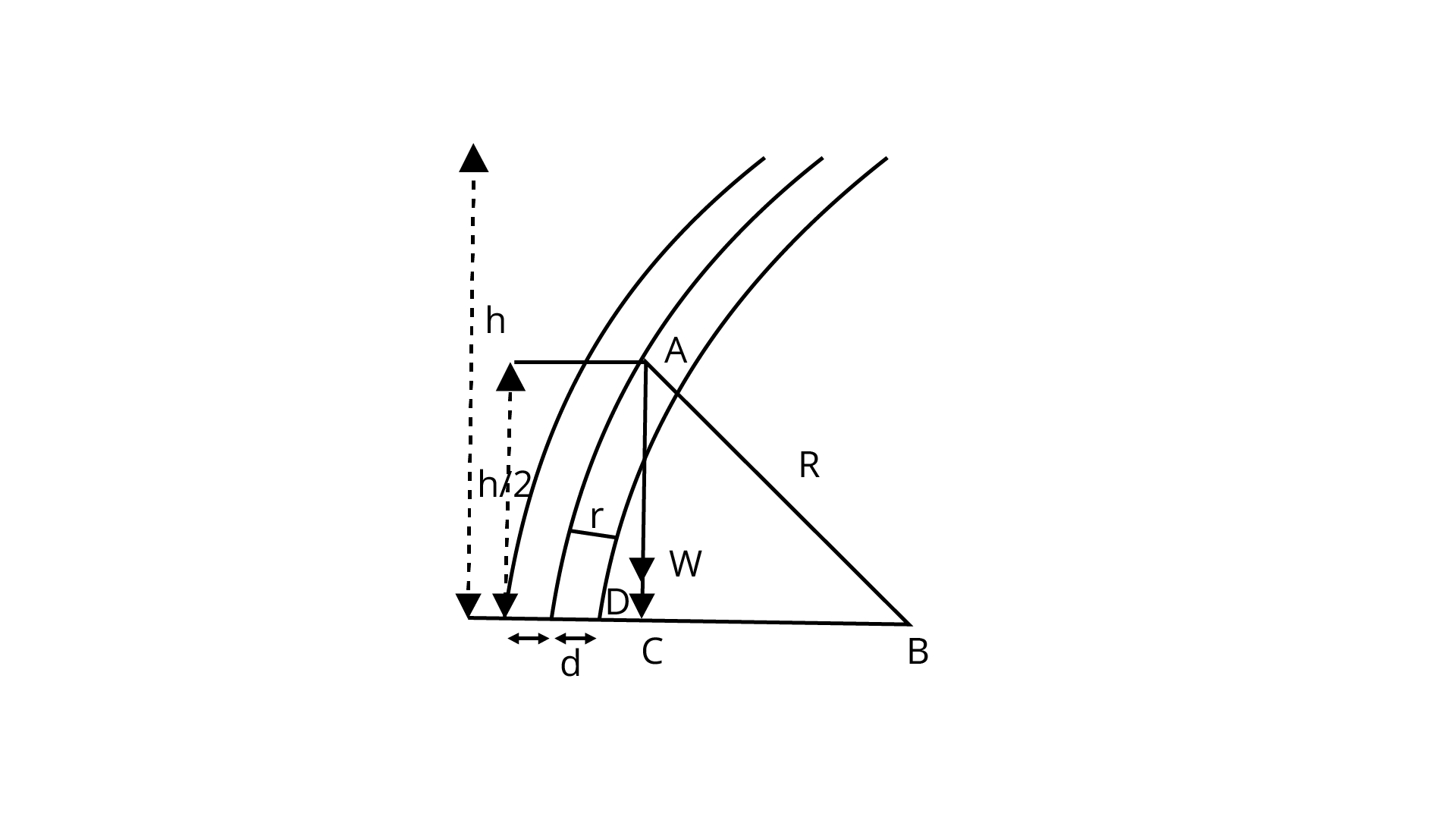${\text{Y}}$ is the Young's modulus, ${\text{r}}$ is the radius of the trunk and ${\text{R}}$ is the radius of curvature of the bent surface along the height of the tree containing the centre of gravity (the neutral surface). Estimate the critical height of a tree for a given radius of the trunk.

Ans: Here we use pythagoras theorem in above figure right angled triangle ABC where C is outside the base of trunk i.e. C is at

${{\text{R}}^{\text{2}}}{\text{ = (R - d}}{{\text{)}}^{\text{2}}}{\text{ + }}{\left( {\dfrac{{\text{h}}}{{\text{2}}}} \right)^{\text{2}}}$

${{\text{R}}^{\text{2}}}{\text{ = }}{{\text{R}}^{\text{2}}}{\text{ + }}{{\text{d}}^{\text{2}}}{\text{ - 2Rd + }}\dfrac{{{{\text{h}}^{\text{2}}}}}{{\text{4}}}$

$\because {\text{ d < < < R}}$

${\text{2Rd = }}\dfrac{{{{\text{h}}^{\text{2}}}}}{{\text{4}}}{\text{ or d = }}\dfrac{{{{\text{h}}^{\text{2}}}}}{{{\text{8R}}}}$                                           (i)

Now, let weight of trunk per unit volume ${\text{ = }}{{\text{W}}_{\text{0}}}$

Weight of trunk ${\text{ = Volume }\times{ }}{{\text{W}}_{\text{0}}}{\text{ = }}\left( {{\text{}\pi{ }}{{\text{r}}^{\text{2}}}{\text{h}}} \right){{\text{W}}_{\text{0}}}$

So, torque by bending trunk ${\text{ = Force}} \bot {\text{distance}}$

${\text{\tau = }\pi{ }}{{\text{r}}^{\text{2}}}{\text{h}}{{\text{W}}_{\text{0}}}{\text{ }\times{ d}}$

${\text{\tau = }}\dfrac{{{\text{}\pi{ }}{{\text{r}}^{\text{4}}}{\text{Y}}}}{{{\text{4R}}}}$

${\text{}\pi{ }}{{\text{r}}^{\text{2}}}{\text{h}}{{\text{W}}_{\text{0}}}{\text{ }\times{ }}\dfrac{{{{\text{h}}^{\text{2}}}}}{{{\text{8R}}}}{\text{ = }}\dfrac{{{\text{}\pi{ }}{{\text{r}}^{\text{4}}}{\text{Y}}}}{{{\text{4R}}}}$

${{\text{h}}^{\text{3}}}{\text{ = }}\dfrac{{{\text{}\pi{ }}{{\text{r}}^{\text{4}}}{\text{Y }\times{ 8R}}}}{{{\text{4R}\pi{ }}{{\text{r}}^{\text{2}}}{{\text{W}}_{\text{0}}}}}{\text{ = }}\dfrac{{{\text{2}}{{\text{r}}^{\text{2}}}{\text{Y}}}}{{{{\text{W}}_{\text{0}}}}}$

${\text{h = }}{\left[ {\dfrac{{{\text{2I}}}}{{{{\text{W}}_{\text{0}}}}}} \right]^{\dfrac{1}{3}}}{{\text{r}}^{\dfrac{2}{3}}}$  which is the critical height in expression

1. A stone of mass ${\text{m}}$ is tied to an elastic string of negligible mass and spring constant ${\text{k}}$. The unstretched length of the string is ${\text{L}}$ and has negligible mass. The other end of the string is fixed to a nail at a point ${\text{P}}$ . Initially the stone is at the same level as the point P. The stone is dropped vertically from point P.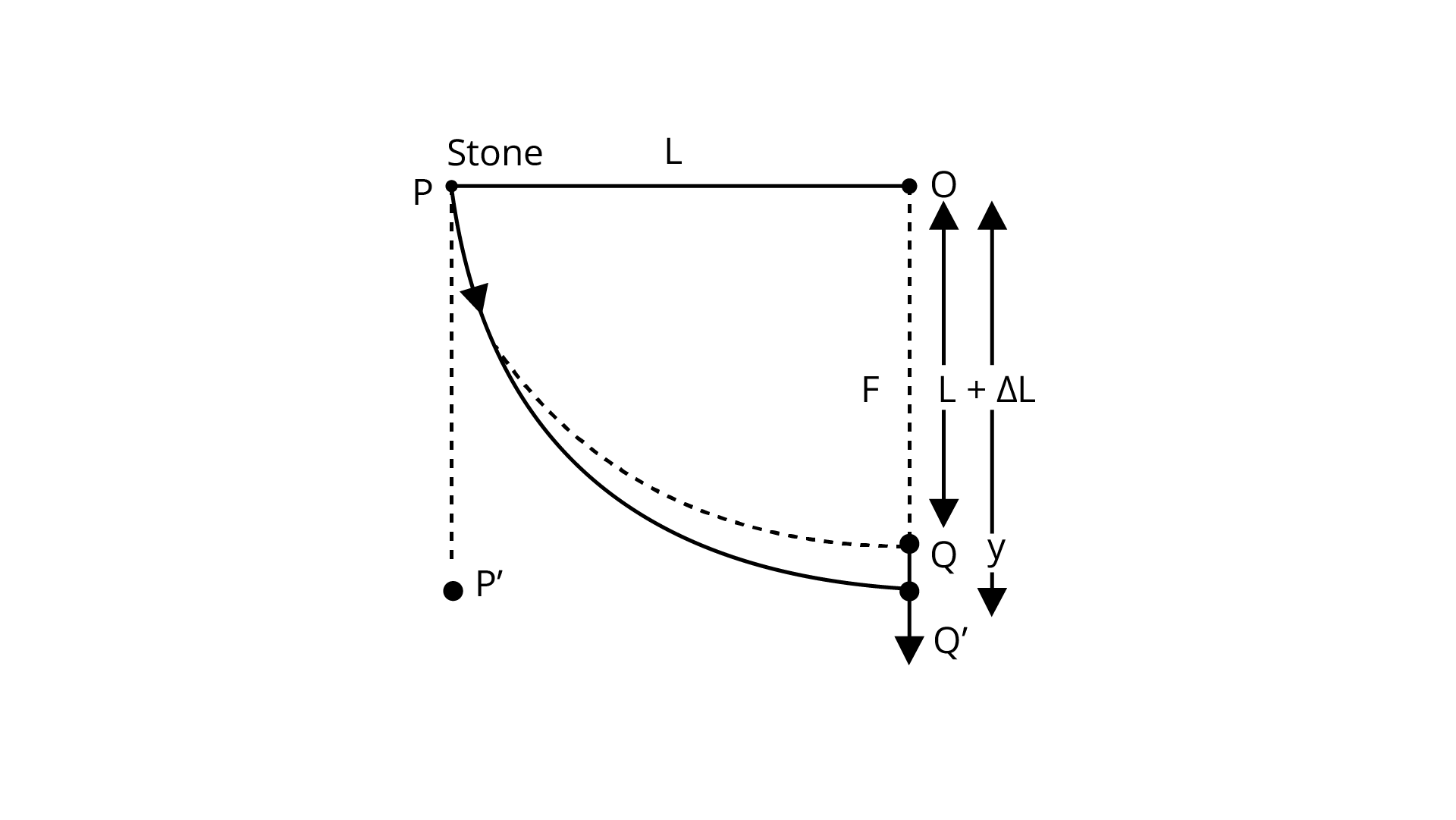(a) Find the distance ${\text{y}}$ from the top when the mass comes to rest for an instant, for the first time.

Ans: Here stone is tied at P with length which denoted as ${\text{L}}$

Here string is fixed at ${\text{O}}$ and stone is lifted with height ${\text{L}}$ as in given figure.

Now, when stone fall under gravity. It follows path PP’ but due to elastic string it will go from P to Q. It is like centrifugal force that stretches string outward and increases its length. Thus, changes in potential energy at ${{\text{Q}}^{\text{'}}}$ and p converts into mechanical energy with ${\text{k}}$

Now, consider P.E. of stone which is equal to mechanical energy

$m g y=\frac{1}{2} K(y-L)^{2}$

$m g y=\frac{1}{2} K\left(y^{2}+L^{2}-2 y L\right)$

$2 m g y=K\left[y^{2}+L^{2}-2 y L\right]$

$2 m g h=K y^{2}-2 K y L+K L^{2}$

$K y^{2}-2 K y L-2 m g y+K L^{2}=0$$K y^{2}-2(K L+m g) y+K L^{2}=0$

Now, solve it by using quadratic formula we get:

$\mathrm{D}=\mathrm{b}^{2}-4 \mathrm{ac} \quad\left(\mathrm{a}=\mathrm{K}, \mathrm{b}=-2(\mathrm{KL}+\mathrm{mg}), \mathrm{c}=\mathrm{KL}^{2}\right)$

$\mathrm{D}=[-2(\mathrm{KL}+\mathrm{mg})]^{2}-4(\mathrm{~K})(\mathrm{KL})^{2}$

$\mathrm{D}=+4\left[(\mathrm{KL})^{2}+(\mathrm{mg})^{2}+2(\mathrm{KL})(\mathrm{mg})\right]-4 \mathrm{~K}^{2} \mathrm{~L}^{2}$

$\mathrm{D}=4\left[\mathrm{~K}^{2} \mathrm{~L}^{2}+\mathrm{m}^{2} \mathrm{~g}^{2}+2 \mathrm{KL} \mathrm{mg}\right]-4 \mathrm{k}^{2} \mathrm{~L}^{2}$

$=4 \mathrm{~K}^{2} \mathrm{~L}^{2}+4 \mathrm{~m}^{2} \mathrm{~g}^{2}+8 \mathrm{KLmg}-4 \mathrm{~K}^{2} \mathrm{~L}^{2}$

$\sqrt{\mathrm{D}}=\sqrt{4 \mathrm{mg}[\mathrm{mg}+2 \mathrm{KL}}=2 \sqrt{\mathrm{mg}(\mathrm{mg}+2 \mathrm{KL})}$

$\mathrm{y}=\frac{-\mathrm{b} \pm \sqrt{\mathrm{D}}}{2 \mathrm{a}}=\frac{+2(\mathrm{KL}+\mathrm{mg} \pm 2 \sqrt{\mathrm{mg}(2 \mathrm{KL}+\mathrm{mg})}}{2 \mathrm{~K}}$

$\mathrm{y}=\frac{2[(\mathrm{KL}+\mathrm{mg})] \pm \sqrt{\mathrm{mg}(2 \mathrm{KL}+\mathrm{m})]}}{2 \mathrm{k}}$

$\mathrm{y}=\frac{(\mathrm{KL}+\mathrm{m} \mathrm{g}) \pm \sqrt{\mathrm{mg}(2 \mathrm{KL}+\mathrm{mg})}}{\mathrm{K}}$

(b) What is the maximum velocity attained by the stone in this drop?

Ans: As on maximum velocity, acceleration is equal to zero

So, string force $\mathrm{kx}$ is balanced by gravitational force and these forces will equal and opposite

$\mathrm{mg}=\mathrm{kx}$

where $x$ represents an extension

Now, let v represents a maximum velocity of stone at the bottom

So, by the law of conservation of energy, we have

K.E. is added with P.E. which is equal to P.E. from $P$ to $Q^{\prime}$

$\frac{1}{2} m v^{2}+\frac{1}{2} K x^{2}=m g(L+x)$

$m v^{2}+K x^{2}=2 m g(L+x)$

$m v^{2}=2 m g L+2 m g x-K x^{2}$

$m g=K x$

$x=\frac{m g}{K}$

$\mathrm{mv}^{2}=2 \mathrm{mgL}+2 \mathrm{mg} \times \frac{\mathrm{mg}}{\mathrm{k}}-\mathrm{K} \frac{\mathrm{m}^{2} \mathrm{~g}^{2}}{\mathrm{~K}^{2}}$

$=2 \mathrm{mgL}+\frac{2 \mathrm{~m}^{2} \mathrm{~g}^{2}}{\mathrm{~K}}-\frac{\mathrm{m}^{2} \mathrm{~g}^{2}}{\mathrm{~K}}$

$\mathrm{mv}^{2}=\mathrm{m}\left[2 \mathrm{gL}+\frac{\mathrm{mg}^{2}}{\mathrm{~K}}\right]$

$\mathrm{v}=\left[2 \mathrm{gL}+\frac{\mathrm{mg}^{2}}{\mathrm{~K}}\right]^{\frac{1}{2}}$

(c) What shall be the nature of the motion after the stone has reached its lowest point?

Ans: From (a)'s lowest point

$\mathrm{F}=\mathrm{mg} \downarrow-\mathrm{k}(\mathrm{y}-\mathrm{L}) \uparrow \quad$ (by string)

$\mathrm{m} \frac{\mathrm{d}^{2} \mathrm{z}}{\mathrm{dt}^{2}}=\mathrm{mg}-\mathrm{K}(\mathrm{y}-\mathrm{L})$ $\frac{\mathrm{d}^{2} \mathrm{z}}{\mathrm{dt}^{2}}-\mathrm{g}+\frac{\mathrm{K}}{\mathrm{m}}(\mathrm{y}-\mathrm{L})=0 \frac{\mathrm{d}^{2} \mathrm{y}}{\mathrm{dt}^{2}}+\frac{\mathrm{K}}{\mathrm{m}}\left[(\mathrm{y}-\mathrm{L})-\frac{\mathrm{mg}}{\mathrm{K}}\right]$

Now, from transformation of variables we have $z=\left[(y-L)-\frac{m g}{K}\right]$

Then $\frac{\mathrm{d}^{2} \mathrm{z}}{\mathrm{dt}^{2}}+\frac{\mathrm{k}}{\mathrm{m}} \mathrm{z}=0$

As this is a differential equation of second order i.e. $\frac{\mathrm{d}^{2} \mathrm{z}}{\mathrm{dt}^{2}}+\omega^{2} z=0$

where $\omega$ is angular frequency so we have $\omega=\sqrt{\frac{\mathrm{K}}{\mathrm{m}}}$

So, solution of differential equation looks like $z=A \cos (\omega t+\theta)$ where $\omega=\sqrt{\frac{\mathrm{K}}{\mathrm{m}}}, \theta$ is phase difference

This implies $z=\left(L+\frac{m}{K} g\right)+A^{\prime} \cos (\omega t+\theta)$

Now, frequency $\omega$ at $\mathrm{y}=0$ we have

$\left|z_{0}\right|=\left|-\left(L+\frac{m g}{K}\right)\right|$

$z_{0}=\left(L+\frac{m g}{K}\right)$

## FAQs on NCERT Exemplar for Class 11 Physics Chapter 9 - Mechanical Properties of Solids (Book Solutions)

1. What are the different Mechanical properties of a particular matter?

The Mechanical properties of particulate matter suggest the various properties of a matter that define the various states of the matter. The concept of the properties of matters has been introduced by CBSE in the syllabus of the students who are preparing for Class 11 and who have opted for Physics as a subject. By studying the Chapters involving the definitions and descriptions of the properties of matter, the students will get to learn about the Elasticity, Stress, Plasticity, and Strain of various matters. Students will learn about various laws that will help them to analyze the concept further.

2. What is suggested by Hooke's law?

This particular law establishes a proven relationship between the stress and strain produced in a particular matter. The law and equation show the direct relationship between the stress and strain considering the constant elastic limit. If the elastic limit is considered to be constant then there can be a direct relationship between the stress and strain which will be directly proportional to each other. In short, if we increase the strain, the stress will also be increased and if we decrease the strain, the stress will also be decreased considering the same elastic limit.

3. Is studying the concept of Mechanical properties important to score well in JEE Main and Advanced?

To qualify for JEE Main or Advanced and to score well in any other Engineering entrance Examination, it is very important for the students that they cover all the topics that have been included in their CBSE syllabus and they go through all the Chapters that are there in the NCERT books. One of the important topics that they have to cover before they appear for the Examinations is the Mechanical properties of different types of matters. It is one of the basic and fundamental concepts of Physics that they need to understand if they want to continue their studies in Physics.

4. What are the study materials that the students should consult to understand the concept of Mechanical properties of Solids?

The NCERT books prescribed by the CBSE are the best study materials that the students can consult. The NCERT Exemplars designed for the Class 11 students can provide them with the detailed guideline of how they should study and what are the important questions that they should consider solving to score well in the Examinations. NCERT Exemplar provides the students with the detailed syllabus that they have to cover and they will get a good idea of all the Chapters from which questions can be there in their Examination.

5. What are the websites to download free study materials for Class 11?

All the important course materials and solved question papers are available on the website of Vedantu. The NCERT Exemplar that is meant for the Class 11 students is available for free on the website and the students can download them in PDF format. Various revision notes and sample question paper sets can help them to get prepared for the Examinations in the most effective and organized way. The questions are solved by learned and experienced trainers who have specialized in their own fields. Also, taking the help of the important notes is highly important for the students because they do not get a lot of time to cover all the Chapters unless they follow a strategic method to study.